This specification defines a core subset of Mathematical Markup Language, or MathML, that is suitable for browser implementation. MathML is a markup language for describing mathematical notation and capturing both its structure and content. The goal of MathML is to enable mathematics to be served, received, and processed on the World Wide Web, just as HTML has enabled this functionality for text.

## Introduction

The [[MATHML3]] specification has several shortcomings that make it hard to implement consistently across web rendering engines or to extend with user-defined constructions, e.g.:

• It is a huge and standalone specification.
• It does not contain any detailed rendering rules.
• It is not driven by browser-implementation.
• It lacks automated testing.

This MathML Core specification intends to address these issues by being as accurate as possible on the visual rendering of mathematical formulas using additional rules from the TeXBook’s Appendix G [[?TEXBOOK]] and from the Open Font Format [[OPEN-FONT-FORMAT]], [[OPEN-TYPE-MATH-ILLUMINATED]]. It also relies on modern browser implementations and web technologies [[HTML]] [[SVG]] [[CSS2]] [[DOM]], clarifying interactions with them when needed or introducing new low-level primitives to improve the web platform layering.

Parts of MathML3 that do not fit well in this framework or are less fundamental have been omitted. Instead, they are described in a separate and larger [[MATHML4]] specification. The details of which math feature will be included in future versions of MathML Core or implemented as polyfills is still open. This question and other potential improvements are tracked on GitHub.

By increasing the level of implementation details, focusing on a workable subset, following a browser-driven design and relying on automated web platform tests, this specification is expected to greatly improve MathML interoperability. Moreover, effort on MathML layering will enable users to implement the rest of the MathML 4 specification, or more generally to extend MathML Core, using modern web technologies such as shadow trees, custom elements or APIs from [[HOUDINI]].

## MathML Fundamentals

### Elements and attributes

The term MathML element refers to any element in the MathML namespace. The MathML elements defined in this specification are called the MathML Core elements and are listed below. Any MathML element that is not listed below is called an Unknown MathML element.

1. [^annotation^]
2. [^annotation-xml^]
3. [^maction^]
4. [^math^]
5. [^merror^]
6. [^mfrac^]
7. [^mi^]
8. [^mmultiscripts^]
9. [^mn^]
10. [^mo^]
11. [^mover^]
13. [^mphantom^]
14. [^mprescripts^]
15. [^mroot^]
16. [^mrow^]
17. [^ms^]
18. [^mspace^]
19. [^msqrt^]
20. [^mstyle^]
21. [^msub^]
22. [^msubsup^]
23. [^msup^]
24. [^mtable^]
25. [^mtd^]
26. [^mtext^]
27. [^mtr^]
28. [^munder^]
29. [^munderover^]
30. [^semantics^]

The grouping elements are [^maction^], [^math^], [^merror^], [^mphantom^], [^mprescripts^], [^mrow^], [^mstyle^], [^semantics^] and unknown MathML elements.

The scripted elements are [^mmultiscripts^], [^mover^], [^msub^], [^msubsup^], [^msup^], [^munder^] and [^munderover^].

The radical elements are [^mroot^] and [^msqrt^].

The attributes defined in this specification have no namespace and are called MathML attributes:

#### The Top-Level [itex] Element

MathML specifies a single top-level or root math element, which encapsulates each instance of MathML markup within a document. All other MathML content must be contained in a [itex] element.

The [itex] element accepts the attributes described in as well as the following attributes:

• [^math/display^]
• [^math/alttext^]

The display attribute, if present, must be an ASCII case-insensitive match to block or inline. The user agent stylesheet described in contains rules for this attribute that affect the default values for the [^math/display^] (block math or inline math) and math-style (normal or compact) properties. If the display attribute is absent or has an invalid value, the User Agent stylesheet treats it the same as inline.

This specification does not define any observable behavior that is specific to the alttext attribute.

The [^math/alttext^] attribute may be used as alternative text by some legacy systems that do not implement math layout.

If the [itex] element does not have its computed [^math/display^] property equal to block math or inline math then it is laid out according to the CSS specification where the corresponding value is described. Otherwise the layout algorithm of the [^mrow^] element is used to produce a math content box. That math content box is used as the content for the layout of the element, as described by CSS for display: block (if the computed value is block math) or display: inline (if the computed value is inline math). Additionally, if the computed [^math/display^] property is equal to block math then that math content box is rendered horizontally centered within the content box.

TEX's display mode $$...$$ and inline mode $...$ correspond to display="block" and display="inline" respectively.

In the following example, a [^math^] formula is rendered in display mode on a new line and taking full width, with the math content centered within the container: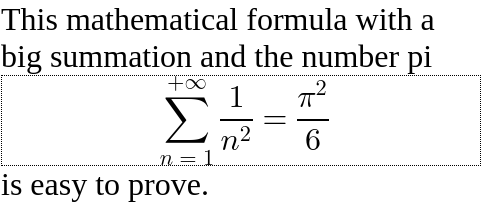As a comparison, the same formula would look as follows in inline mode. The formula is embedded in the paragraph of text without forced line breaking. The baselines specified by the layout algorithm of the [^mrow^] are used for vertical alignment. Note that the middle of sum and equal symbols or fractions are all aligned, but not with the alphabetical baseline of the surrounding text.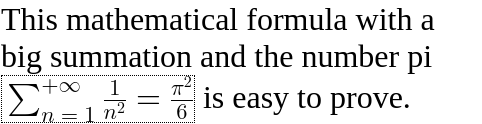Because good mathematical rendering requires use of mathematical fonts, the user agent stylesheet should set the font-family to the math value on the [itex] element instead of inheriting it. Additionally, several CSS properties that can be set on a parent container such as font-style, font-weight, direction or text-indent etc are not expected to apply to the math formula and so the user agent stylesheet has rules to reset them by default.

#### Types for MathML Attribute Values

In addition to CSS data types, some MathML attributes rely on the following MathML-specific types:

unsigned-integer
An <integer> value as defined in [[CSS-VALUES-4]], whose first character is neither U+002D HYPHEN-MINUS character (-) nor U+002B PLUS SIGN (+).
boolean
A string that is an ASCII case-insensitive match to true or false.

#### Global Attributes

The following attributes are common to and may be specified on all MathML elements:

#### Attributes common to HTML and MathML elements

The id, class, style, data-*, nonce and tabindex attributes have the same syntax and semantics as defined for [^global/id^], [^global/class^], [^html-global/style^], data-*, [^htmlsvg-global/nonce^] and [^htmlsvg-global/tabindex^] attributes on HTML elements.

The dir attribute, if present, must be an ASCII case-insensitive match to ltr or rtl. In that case, the user agent is expected to treat the attribute as a presentational hint setting the element's direction property to the corresponding value. More precisely, an ASCII case-insensitive match to rtl is mapped to rtl while an ASCII case-insensitive match to ltr is mapped to ltr.

The dir attribute is used to set the directionality of math formulas, which is often rtl in Arabic speaking world. However, languages written from right to left often embed math written from left to right and so the user agent stylesheet resets the direction property accordingly on the [^math^] elements.

In the following example, the dir attribute is used to render "𞸎 plus 𞸑 raised to the power of (٢ over, 𞸟 plus ١)" from right-to-left.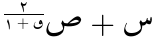All MathML elements support event handler content attributes, as described in event handler content attributes in HTML.

All event handler content attributes noted by HTML as being supported by all HTMLElements are supported by all MathML elements as well, as defined in the MathMLElement IDL.

#### Legacy MathML Style Attributes

The mathcolor and mathbackground attributes, if present, must have a value that is a <color>. In that case, the user agent is expected to treat these attributes as a presentational hint setting the element's color and background-color properties to the corresponding values. The mathcolor attribute describes the foreground fill color of MathML text, bars etc while the mathbackground attribute describes the background color of an element.

The mathsize attribute, if present, must have a value that is a valid <length-percentage>. In that case, the user agent is expected to treat the attribute as a presentational hint setting the element's font-size property to the corresponding value. The mathsize property indicates the desired height of glyphs in math formulas but also scales other parts (spacing, shifts, line thickness of bars etc) accordingly.

The above attributes are implemented for compatibility with full MathML. Authors whose only target is MathML Core are encouraged to use CSS for styling.

#### The displaystyle and scriptlevel attributes

The displaystyle attribute, if present, must have a value that is a boolean. In that case, the user agent is expected to treat the attribute as a presentational hint setting the element's math-style property to the corresponding value. More precisely, an ASCII case-insensitive match to true is mapped to normal while an ASCII case-insensitive match to false is mapped to compact. This attribute indicates whether formulas should try to minimize the logical height (value is false) or not (value is true) e.g. by changing the size of content or the layout of scripts.

The scriptlevel attribute, if present, must have value +<U>, -<U> or <U> where <U> is an unsigned-integer. In that case the user agent is expected to treat the scriptlevel attribute as a presentational hint setting the element's math-depth property to the corresponding value. More precisely, +<U>, -<U> and <U> are respectively mapped to add(<U>) add(<-U>) and <U>.

displaystyle and scriptlevel values are automatically adjusted within MathML elements. To fully implement these attributes, additional CSS properties must be specified in the user agent stylesheet as described in . In particular, for all MathML elements a default font-size: math is specified to ensure that scriptlevel changes are taken into account.

In this example, an [^munder^] element is used to attach a script "A" to a base "∑". By default, the summation symbol is rendered with the font-size inherited from its parent and the A as a scaled down subscript. If displaystyle is true, the summation symbol is drawn bigger and the "A" becomes an underscript. If scriptlevel is reset to 0 on the "A", then it will use the same font-size as the top-level math root.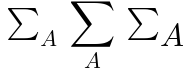TEX's \displaystyle, \textstyle, \scriptstyle, and \scriptscriptstyle correspond to displaystyle and scriptlevel as true and 0, false and 0, false and 1, and false and 2, respectively.

#### Attributes Reserved as Valid

The attributes intent and arg are reserved as valid attributes.

This specification does not define any observable behavior that is specific to the intent and arg attributes.

These attributes are described in [[MATHML4]] and future versions of this specification may or may not define them. Authors should be aware that they are currently in development and subject to change.

### Integration in the Web Platform

#### HTML and SVG

When parsing HTML documents user agents must treat any tag name corresponding to a MathML Core Element as belonging to the MathML namespace.

Users agents must allow mixing HTML, SVG and MathML elements as allowed by sections HTML integration point, MathML integration point, tree construction dispatcher, MathML and SVG from [[HTML]].

When evaluating the SVG [^svg/requiredExtensions^] attribute, user agents must claim support for the language extension identified by the MathML namespace.

In this example, inline MathML and SVG elements are used inside an HTML document. SVG elements <switch> and <foreignObject> (with proper <requiredExtensions>) are used to embed a MathML formula with a text fallback, inside a diagram. HTML input element is used within the [^mtext^] to include an interactive input field inside a mathematical formula.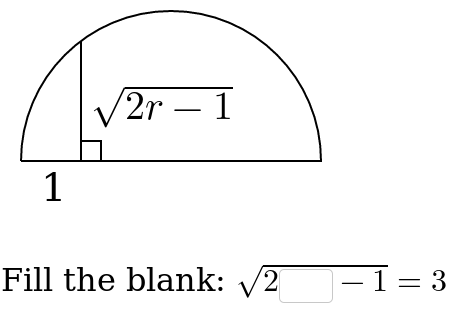• The [itex] element can be used at position permitted for flow content (e.g. a <foreignObject> element) or phrasing content.
• Any phrasing content can be used inside [^mi^], [^mo^], [^mn^], [^ms^] and [^mtext^] elements.
• The <svg> element can be used inside [^annotation-xml^] elements.
• Any flow content can be used inside [^annotation-xml^] elements with encoding application/xhtml+xml or text/html.

#### CSS styling

User agents must support various CSS features mentioned in this specification, including new ones described in . They must follow the computation rule for display: contents.

In this example, the MathML formula inherits the CSS color of its parent and uses the font-family specified via the style attribute.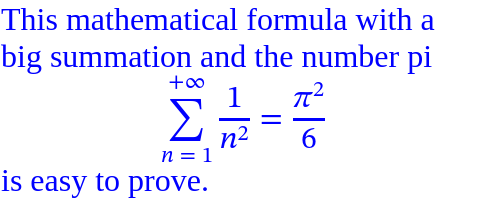All documents containing MathML Core elements must include CSS rules described in as part of user-agent level style sheet defaults. In particular, this adds !important rules to force writing mode to horizontal-lr on all MathML elements.

The float property does not create floating of elements whose parent's computed display value is block math or inline math, and does not take them out-of-flow.

The following CSS features are not supported and must be ignored:

• Line breaking inside math formulas: white-space is treated as nowrap on all MathML elements.
• Alignment properties: align-content, justify-content, align-self, justify-self have no effects on MathML elements.
These features might be handled in future versions of this document. For now, authors are discouraged from setting a different value for these properties as that might lead to backward incompatibility issues.

#### DOM and JavaScript

User agents supporting Web application APIs must ensure that they keep the visual rendering of MathML synchronized with the [[DOM]] tree, in particular perform necessary updates when MathML attributes are modified dynamically.

All the nodes representing MathML elements in the DOM must implement, and expose to scripts, the following MathMLElement interface.

The {{GlobalEventHandlers}} and HTMLOrForeignElement interfaces are defined in [[HTML]].

Each IDL attribute of the MathMLElement interface [=Attr/reflects=] the corresponding MathML content attribute.

In the following example, a MathML formula is used to render the fraction "α over 2". When clicking the red α, it is changed into a blue β.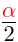Rename HTMLOrSVGElement and define MathMLElement in [[HTML]].

#### Text layout

Because math fonts generally contain very tall glyphs such as big integrals, using typographic metrics is important to avoid excessive line spacing of text. As a consequence, user agents must take into account the USE_TYPO_METRICS flag from the OS/2 table [[OPEN-FONT-FORMAT]] when performing text layout.

#### Focus

MathML provides the ability for authors to allow for interactivity in supporting interactive user agents using the same concepts, approach and guidance to Focus as described in HTML, with modifications or clarifications regarding application for MathML as described in this section.

When an element is focused, all applicable CSS focus-related pseudo-classes as defined in Selectors Level 3 apply, as defined in that specification.

The contents of embedded [^math^] elements (including HTML elements inside token elements) contribute to the sequential focus order of the containing owner HTML document (combined sequential focus order).

## Presentation Markup

### Visual formatting model

#### Box Model

The default [^math/display^] property is described in :

• For the [itex] root, it is equal to inline math or block math according to the value of the [^math/display^] attribute.
• For Tabular MathML elements [^mtable^], [^mtr^], [^mtd^] it is respectively equal to inline-table, table-row and table-cell.
• For all but the first children of the [^maction^] and [^semantics^] elements, it is equal to none.
• For all the other MathML elements it is equal to block math.

In order to specify math layout in different writing modes, this specification uses concepts from [[CSS-WRITING-MODES-4]]:

Unless specified otherwise, the figures in this specification use a writing mode of horizontal-lr and ltr. See , and for examples of other writing modes that are sometimes used for math layout.

Boxes used for MathML elements rely on several parameters in order to perform layout in a way that is compatible with CSS but also to take into account very accurate positions and spacing within math formulas:

1. Inline metrics. min-content inline size, max-content inline size and inline size from CSS. See .Generic Box Model for MathML elements
2. Block metrics. The block size, first baseline set and last baseline set. The following baselines are defined for MathML boxes:

1. The alphabetic baseline which typically aligns with the bottom of uppercase Latin glyphs. The algebraic distance from the alphabetic baseline to the line-over edge of the box is called the line-ascent. The algebraic distance from the line-under edge to the alphabetic baseline of the box is called the line-descent.
2. The mathematical baseline, also called math axis, which typically aligns with the fraction bar, middle of fences and binary operators. It is shifted away from the alphabetic baseline by AxisHeight towards the line-over.
3. The ink-over baseline, indicating the line-over theorical limit of the math content drawn, excluding any extra space. If not specified, it is aligned with the line-over edge. The algebraic distance from the alphabetic baseline to the ink-over baseline is called the ink line-ascent.
4. The ink-under baseline, indicating the line-under theorical limit of the math content drawn, excluding any extra space. If not specified, it is aligned with the line-under edge. The algebraic distance from the ink-under baseline to the alphabetic baseline is called the ink line-descent.
For math layout, it is very important to rely on the ink extent when positioning text. This is not the case for more complex notations (e.g. square root). Although ink-ascent and ink-descent are defined for all MathML elements they are really only used for the token elements. In other cases, they just match normal ascent and descent.
Unless specified otherwise, the last baseline set is equal to the first baseline set for MathML boxes.
3. An optional italic correction which provides a measure of how much the text of a box is slanted along the inline axis. See .Examples of italic correction for italic f and large integral If it is requested during calculation of min-content inline size and max-content inline size or during layout then 0 is used as a fallback value.
4. An optional top accent attachment which provides a reference offset on the inline axis of a box that should be used when positioning that box as an accent. See .Example of top accent attachment for a circumflex accent If it is requested during calculation of min-content inline size (respectively max-content inline size) then half the min-content inline size (respectively max-content inline size) is used as a fallback value. If it is requested during layout then half the inline size of the box is used as a fallback value.

Given a MathML box, the following offsets are defined:

• The inline offset of a child box is the offset between the inline-start edge of the parent box and the inline-start edge of the child box.
• The block offset of a child box is the offset between the block-start edge of the parent box and the block-start edge of the child box.
• The line-left offset of a child box is the offset between the line-left edge of the parent box and the line-left edge of the child box.Box model for writing mode horizontal-tb and rtl that may be used in e.g. Arabic math.Box model for writing mode vertical-lr and ltr that may be used in e.g. Mongolian math.Box model for writing mode vertical-rl and ltr that may be used in e.g. Japanese math.
The position of child boxes and graphical items inside a MathML box are expressed using the inline offset and block offset. For convenience, the layout algorithms may describe offsets using flow-relative directions, line-relative directions or the alphabetic baseline. It is always possible to pass from one description to the other because position of child boxes is always performed after the metrics of the box and of its child boxes are calculated.

Here are examples of offsets obtained from line-relative metrics:

Improve definition of ink ascent/descent?

#### Layout Algorithms

Each MathML element has an associated math content box, which is calculated as described in this chapter's layout algorithms using the following structure:

1. Calculation of min-content inline size and max-content inline size of the math content.
2. Box layout:
1. Layout of in-flow child boxes.
2. Calculation of inline size, ink line-ascent, ink line-descent, line-ascent and line-ascent of the math content.
3. Calculation of offsets of child boxes within the math content box as well as sizes and offsets of extra graphical items (bars, radical symbol, etc).
4. Layout and positioning of absolutely-positioned and fixed-positioned boxes, as described in [[CSS-POSITION-3]].

The following extra steps must be performed:

Per the description above, margin-collapsing does not apply to MathML elements.

During box layout, optional inline stretch size constraint and block stretch size constraint parameters may be used on embellished operators. The former indicates a target size that a [=embellished operator/core operator=] stretched along the inline axis should cover. The latter indicates an ink line-ascent and ink line-descent that a [=embellished operator/core operator=] stretched along the block axis should cover. Unless specified otherwise, these parameters are ignored during box layout and child boxes are laid out without any stretch size constraint.

Define what inline percentages resolve against
Define what block percentages resolve against

#### Anonymous <mrow> boxes

An anonymous box is a box without any associated element in the DOM tree and which is generated for layout purpose only. The properties of anonymous boxes are inherited from the enclosing non-anonymous box while non-inherited properties have their initial value. An anonymous <mrow> box is an anonymous box with display equal to block math and which is laid out as described in section .

If a MathML element generates an anonymous <mrow> box if it wraps in children in an anonymous <mrow> box i.e. its subtree in the visual formatting model is made of an anonymous <mrow> box which itself contains the boxes associated to the children of this MathML element.

In the following example, the [^math^] and [^mrow^] elements are laid out as described in section . In particular, the [itex] element adds proper spacing around its <mo>≠</mo> child and the <mrow> element stretches its <mo>|</mo> children vertically.

The [^mtd^] element has display: table-cell and the [^msqrt^] element displays a radical symbol around its children. However, they also place their children in a way that is similar to what is described in section : the <msqrt> element adds proper spacing around its <mo>+</mo> child while the <mtd> element stretches its <mo> children vertically. In order to make this possible, each of these two elements generates an anonymous <mrow> box.#### Stacking contexts

MathML elements can overlap due to various spacing rules. They can as well contain extra graphical items (bars, radical symbol, etc). A MathML element with computed style display: block math or display: inline math generates a new stacking context. The painting order of in-flow children of such a MathML element is exactly the same as block elements. The extra graphical items are painted after text and background (right after step 7.2.4 for display: inline math and right after step 7.2 for display: block math).

### Token Elements

Token elements in presentation markup are broadly intended to represent the smallest units of mathematical notation which carry meaning. Tokens are roughly analogous to words in text. However, because of the precise, symbolic nature of mathematical notation, the various categories and properties of token elements figure prominently in MathML markup. By contrast, in textual data, individual words rarely need to be marked up or styled specially.

In practice, most MathML token elements just contain simple text for variables, numbers, operators etc and don't need sophisticated layout. However, it can contain text with line breaks or arbitrary HTML5 phrasing elements.

#### Text <mtext>

The mtext element is used to represent arbitrary text that should be rendered as itself. In general, the <mtext> element is intended to denote commentary text.

The <mtext> element accepts the attributes described in .

In the following example, [^mtext^] is used to put conditional words in a definition: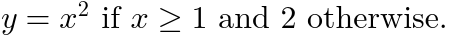#### Layout of <mtext>

If the element does not have its computed [^math/display^] property equal to block math or inline math then it is laid out according to the CSS specification where the corresponding value is described. Otherwise, the layout below is performed.

If the <mtext> element contains only text content without forced line break or soft wrap opportunity then, the anonymous child node generated for that text is laid out as defined in the relevant CSS specification and:

Otherwise, the mtext element is laid out as a block box and corresponding min-content inline size, max-content inline size, inline size, block size, first baseline set and last baseline set are used for the math content box.

#### Identifier <mi>

The mi element represents a symbolic name or arbitrary text that should be rendered as an identifier. Identifiers can include variables, function names, and symbolic constants.

The <mi> element accepts the attributes described in as well as the following attribute:

• [^mi/mathvariant^]

The layout algorithm is the same as the [^mtext^] element. The user agent stylesheet must contain the following property in order to implement automatic italic via the text-transform value introduced in :

The mathvariant attribute, if present, must be an ASCII case-insensitive match of normal. In that case, the user agent is expected to treat the attribute as a presentational hint setting the element's text-transform property to none. Otherwise it has no effects.

In [[MathML3]], the mathvariant attribute was used to define logical classes of token elements, each class providing a collection of typographically-related symbolic tokens with specific meaning within a given mathematical expression.

In MathML Core, this attribute is only used to cancel automatic italic of the [^mi^] element. For other use cases, the proper Mathematical Alphanumeric Symbols [[UNICODE]] should be used instead. See also section .

In the following example, [^mi^] is used to render variables and function names. Note that identifiers containing a single letter are italic by default.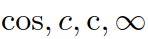#### Number <mn>

The mn element represents a "numeric literal" or other data that should be rendered as a numeric literal. Generally speaking, a numeric literal is a sequence of digits, perhaps including a decimal point, representing an unsigned integer or real number.

The <mn> element accepts the attributes described in . Its layout algorithm is the same as the [^mtext^] element.

In the following example, [^mn^] is used to write a decimal number.#### Operator, Fence, Separator or Accent <mo>

The mo element represents an operator or anything that should be rendered as an operator. In general, the notational conventions for mathematical operators are quite complicated, and therefore MathML provides a relatively sophisticated mechanism for specifying the rendering behavior of an <mo> element.

As a consequence, in MathML the list of things that should "render as an operator" includes a number of notations that are not mathematical operators in the ordinary sense. Besides ordinary operators with infix, prefix, or postfix forms, these include fence characters such as braces, parentheses, and "absolute value" bars; separators such as comma and semicolon; and mathematical accents such as a bar or tilde over a symbol. This chapter uses the term "operator" to refer to operators in this broad sense.

The <mo> element accepts the attributes described in as well as the following attributes:

• [^mo/form^]
• [^mo/fence^]
• [^mo/separator^]
• [^mo/lspace^]
• [^mo/rspace^]
• [^mo/stretchy^]
• [^mo/symmetric^]
• [^mo/maxsize^]
• [^mo/minsize^]
• [^mo/largeop^]
• [^mo/movablelimits^]

This specification does not define any observable behavior that is specific to the fence and separator attributes.

Authors may use the [^mo/fence^] and [^mo/separator^] to describe specific semantics of operators. The default values may be determined from the Operators_fence and Operators_separator tables, or equivalently the human-readable version of the operator dictionary.

In the following example, the [^mo^] element is used for the binary operator +. Default spacing is symmetric around that operator. A tigher spacing is used if you rely on the form attribute to force it to be treated as a prefix operator. Spacing can also be specified explicitly using the [^mo/lspace^] and [^mo/rspace^] attributes.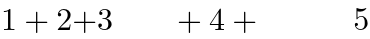Another use case is for big operators such as summation. When displaystyle is true, such an operator is drawn larger but one can change that with the [^mo/largeop^] attribute. When displaystyle is false, underscripts are actually rendered as subscripts but one can change that with the [^mo/movablelimits^] attribute.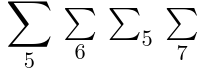Operators are also used for stretchy symbols such as fences, accents, arrows etc. In the following example, the vertical arrow stretches to the height of the [^mspace^] element. One can override default stretch behavior with the [^mo/stretchy^] attribute e.g. to force an unstretched arrow. The [^mo/symmetric^] attribute allows to indicate whether the operator should stretch symmetrically above and below the math axis (fraction bar). Finally the [^mo/minsize^] and [^mo/maxsize^] attributes add additional constraints over the stretch size.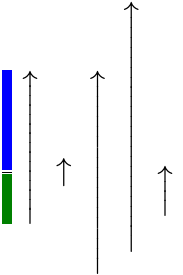Note that the default properties of operators are dictionary-based, as explained in . For example a binary operator typically has default symmetric spacing around it while a fence is generally stretchy by default.

##### Embellished operators

A MathML Core element is an embellished operator if it is:

1. An [^mo^] element;
2. a scripted element or an [^mfrac^], whose first in-flow child exists and is an embellished operator;
3. a grouping element or [^mpadded^], whose in-flow children consist (in any order) of one embellished operator and zero or more space-like elements.

The core operator of an embellished operator is the <mo> element defined recursively as follows:

1. The core operator of an [^mo^] element; is the element itself.
2. The core operator of an embellished scripted element or [^mfrac^] element is the core operator of its first in-flow child.
3. The core operator of an embellished grouping element or [^mpadded^] is the core operator of its unique embellished operator in-flow child.

The stretch axis of an embellished operator is inline if its [=embellished operator/core operator=] contains only text content made of a single character c, and that character has inline intrinsic stretch axis. Otherwise, the stretch axis of the embellished operator is block.

The same definitions apply for boxes in the visual formatting model where an anonymous <mrow> box is treated as a grouping element.

#### Dictionary-based attributes

The form property of an embellished operator is either infix, prefix or postfix. The corresponding form attribute on the [^mo^] element, if present, must be an ASCII case-insensitive match to one of these values.

The algorithm for determining the form of an embellished operator is as follows:

1. If the form attribute is present and valid on the [=embellished operator/core operator=], then its ASCII lowercased value is used.
2. If the embellished operator is the first in-flow child of a grouping element, [^mpadded^] or [^msqrt^] with more than one in-flow child (ignoring all space-like children) then it has form prefix.
3. Or, if the embellished operator is the last in-flow child of a grouping element, [^mpadded^] or [^msqrt^] with more than one in-flow child (ignoring all space-like children) then it has form postfix.
4. Or, if the embellished operator is an in-flow child of a scripted element, other than the first in-flow child, then it has form postfix.
5. Otherwise, the embellished operator has form infix.

The stretchy, symmetric, largeop, movablelimits properties of an embellished operator are either false or true. In the latter case, it is said that the embellished operator has the property. The corresponding stretchy, symmetric, largeop, movablelimits attributes on the [^mo^] element, if present, must be a boolean.

The lspace, rspace, minsize properties of an embellished operator are <length-percentage>. The maxsize property of an embellished operator is either a <length-percentage> or ∞. The lspace, rspace, minsize and maxsize attributes on the [^mo^] element, if present, must be a <length-percentage>.

The algorithm for determining the properties of an embellished operator is as follows:

1. If the corresponding stretchy, symmetric, largeop, movablelimits, lspace, rspace, maxsize or minsize attribute is present and valid on the [=embellished operator/core operator=], then the ASCII lowercased value of this property is used.
2. Otherwise, run the algorithm for determining the form of an embellished operator.
3. If the [=embellished operator/core operator=] contains only text content Content, then set Category to the result of the algorithm to determine the category of an operator (Content, Form) where Form is the form calculated at the previous step.
4. If Category is Default and the form of embellished operator was not explicitly specified as an attribute on its [=embellished operator/core operator=]:
1. Set Category to the result of the algorithm to determine the category of an operator (Content, Form) where Form is infix.
2. If Category is Default, then run the algorithm again with Form set to postfix.
3. If Category is Default, then run the algorithm again with Form set to prefix.
5. Run the algorithm to set the properties of an operator from its category Category.

When used during layout, the values of [=embellished operator/stretchy=], [=embellished operator/symmetric=], [=embellished operator/largeop=], [=embellished operator/movablelimits=], [=embellished operator/lspace=], [=embellished operator/rspace=], [=embellished operator/minsize=] are obtained by the algorithm for determining the properties of an embellished operator with the following extra resolutions:

• Percentage values for lspace, rspace are interpreted relative to the value read from the dictionary or to the fallback value above.
• Interpretation of percentage values for minsize and maxsize are described in .
• Font-relative lengths for lspace, rspace, minsize and maxsize rely on the font style of the [=embellished operator/core operator=], not the one of the embellished operator.

#### Layout of operators

If the <mo> element does not have its computed [^math/display^] property equal to block math or inline math then it is laid out according to the CSS specification where the corresponding value is described. Otherwise, the layout below is performed.

The text of the operator must only be painted if the visibility of the <mo> element is visible. In that case, it must be painted with the color of the <mo> element.

Operators are laid out as follows:

1. If the content of the <mo> element is not made of a single character c then fall back to the layout algorithm of .
2. If the operator has the [=embellished operator/stretchy=] property:
• If the [=embellished operator/stretch axis=] of the operator is inline:
1. If it is not possible to shape a stretchy glyph corresponding to c in the inline direction with the first available font then fall back to the layout algorithm of .
2. The min-content inline size and max-content inline size of the math content are set to the one obtained by the layout algorithm of .
3. If there is not any inline stretch size constraint Tinline then fall back to the layout algorithm of .
4. The inline size and (ink) block metrics of the math content are given by algorithm to shape a stretchy glyph to inline dimension Tinline.
5. The painting of the operator is performed by the algorithm to shape a stretchy glyph stretched to inline dimension Tinline and at position determined by the previous box metrics.
• Otherwise, the [=embellished operator/stretch axis=] of the operator is block. The following steps are performed:
1. If it is not possible to shape a stretchy glyph corresponding to c in the block direction with the first available font then fall back to the layout algorithm of .
2. The min-content inline size and max-content inline size of the math content are set to the preferred inline size of a glyph stretched along the block axis.
3. If there is not any block stretch size constraint (Uascent, Udescent) then fall back to the layout algorithm of .
4. If the operator has the [=embellished operator/symmetric=] property then set the target sizes Tascent and Tdescent to Sascent and Sdescent respectively: Otherwise set them to Uascent and Udescent respectively.
5. Let minsize and maxsize be the [=embellished operator/minsize=] and [=embellished operator/maxsize=] properties on the operator. Percentage values are interpreted relative to T = Tascent + Tdescent. If minsize < 0 then set minsize to 0. If maxsize < minsize then set maxsize to minsize. If 0 ≤ minsizemaxsize:
• If T ≤ 0 then set Tascent to minsize / 2 and then set Tdescent to minsizeTascent.
• Otherwise, if 0 < T < minsize then first multiply Tascent by minsize / T and then set Tdescent to minsize - Tascent.
• Otherwise, if maxsize < T then first multiply Tascent by maxsize / T and then set Tdescent to maxsizeTascent.
6. The inline size, ink line-ascent, ink line-descent, line-ascent and line-descent of the math content are obtained by the algorithm to shape a stretchy glyph to block dimension Tascent + Tdescent. The inline size of the math content is the width of the stretchy glyph. The stretchy glyph is shifted towards the line-under by a value Δ so that its center aligns with the center of the target: the ink ascent of the math content is the ascent of the stretchy glyph − Δ and the ink descent of the math content is the descent of the stretchy glyph + Δ. These centers have coordinates "½(ascent − descent)" so Δ = [(ascent of stretchy glyph − descent of stretchy glyph) − (TascentTdescent)] / 2.
7. The painting of the operator is performed by the algorithm to shape a stretchy glyph stretched to block dimension Tascent + Tdescent and at position determined by the previous box metrics shifted by Δ towards the line-over.Base size, size variants and glyph assembly for the left brace
3. If the operator has the [=embellished operator/largeop=] property and if math-style on the <mo> element is normal, then:
1. Use the MathVariants table to try and find a glyph of height at least DisplayOperatorMinHeight. If none is found, fall back to the largest non-base glyph. If none is found, fall back to the layout algorithm of .

2. The min-content inline size, max-content inline size, inline size and block metrics of the math content are given by the glyph found.
3. Paint the glyph.
4. Otherwise fall back to the layout algorithm of .

If the algorithm to shape a stretchy glyph has been used for one of the step above, then the italic correction of the math content is set to the value returned by that algorithm.

If maxsize is equal to its default value ∞ then minsize ≤ maxsize is satisfied but maxsize < T is not.

#### Space <mspace>

The mspace empty element represents a blank space of any desired size, as set by its attributes.

The <mspace> element accepts the attributes described in as well as the following attributes:

• [^mspace/width^]
• [^mspace/height^]
• [^mspace/depth^]

The width, height, depth, if present, must have a value that is a valid <length-percentage>.

• If the width attribute is present, valid and not a percentage then that attribute is used as a presentational hint setting the element's width property to the corresponding value.
• If the height attribute is absent, invalid or a percentage then the requested line-ascent is 0. Otherwise the requested line-ascent is the resolved value of the height attribute, clamping negative values to 0.
• If both the height and depth attributes are present, valid and not a percentage then they are used as a presentational hint setting the element's height property to the concatenation of the strings "calc(", the height attribute value, " + ", the depth attribute value, and ")". If only one of these attributes is present, valid and not a percentage then it is treated as a presentational hint setting the element's height property to the corresponding value.

In the following example, [^mspace^] is used to force spacing within the formula (a 1px blue border is added to easily visualize the space):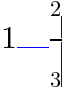If the <mspace> element does not have its computed [^math/display^] property equal to block math or inline math then it is laid out according to the CSS specification where the corresponding value is described. Otherwise, the <mspace> element is laid out as shown on . The min-content inline size, max-content inline size and inline size of the math content are equal to the resolved value of the width property. The block size of the math content is equal to the resolved value of the height property. The line-ascent of the math content is equal to the requested line-ascent determined above.

The terminology height/depth comes from [[MATHML3]], itself inspired from [[TEXBOOK]].

### Definition of space-like elements

A number of MathML presentation elements are "space-like" in the sense that they typically render as whitespace, and do not affect the mathematical meaning of the expressions in which they appear. As a consequence, these elements often function in somewhat exceptional ways in other MathML expressions.

A MathML Core element is a space-like element if it is:

1. an [^mtext^] or [^mspace^];
2. or a grouping element or [^mpadded^] all of whose in-flow children are space-like.

The same definitions apply for boxes in the visual formatting model where an anonymous <mrow> box is treated as a grouping element.

Note that an [^mphantom^] is not automatically defined to be space-like, unless its content is space-like. This is because operator spacing is affected by whether adjacent elements are space-like. Since the <mphantom> element is primarily intended as an aid in aligning expressions, operators adjacent to an <mphantom> should behave as if they were adjacent to the contents of the <mphantom>, rather than to an equivalently sized area of whitespace.

#### String Literal <ms>

ms element is used to represent "string literals" in expressions meant to be interpreted by computer algebra systems or other systems containing "programming languages".

The <ms> element accepts the attributes described in . Its layout algorithm is the same as the [^mtext^] element.

In the following example, [^ms^] is used to write a literal string of characters: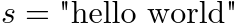In MathML3, it was possible to use the lquote and rquote attributes to respectively specify the strings to use as opening and closing quotes. These are no longer supported and the quotes must instead be specified as part of the text of the <ms> element. One can add CSS rules to legacy documents in order to preserve visual rendering. For example, in left-to-right direction:

### General Layout Schemata

Besides tokens there are several families of MathML presentation elements. One family of elements deals with various "scripting" notations, such as subscript and superscript. Another family is concerned with matrices and tables. The remainder of the elements, discussed in this section, describe other basic notations such as fractions and radicals, or deal with general functions such as setting style properties and error handling.

#### Group Sub-Expressions <mrow>

The mrow element is used to group together any number of sub-expressions, usually consisting of one or more <mo> elements acting as "operators" on one or more other expressions that are their "operands".

In the following example, [^mrow^] is used to group a sum "1 + 2/3" as a fraction numerator (first child of [^mfrac^]) and to construct a fenced expression (first child of [^msup^]) that is raised to the power of 5. Note that [^mrow^] alone does not add visual fences around its grouped content, one has to explicitly specify them using the [^mo^] element.

Within the [^mrow^] elements, one can see that vertical alignment of children (according to the alphabetic baseline or the mathematical baseline) is properly performed, fences are vertically stretched and spacing around the binary + operator automatically calculated.The <mrow> element accepts the attributes described in . An <mrow> element with in-flow children child1, child2, …, childN is laid out as shown on . The child boxes are put in a row one after the other with all their alphabetic baselines aligned.

Because the box model ensures alignment of alphabetic baselines, fraction bars or symmetric stretchy operators will also be aligned along the math axis in the typical case when AxisHeight is the same for all in-flow children.
##### Algorithm for stretching operators along the block axis

The algorithm for stretching operators along the block axis consists in the following steps:

1. If there is a block stretch size constraint or an inline stretch size constraint then the element being laid out is an embellished operator. Lay out the one in-flow child that is an embellished operator with the same stretch size constraint and all the other in-flow children without any stretch size constraint and stop.
2. Otherwise, split the list of in-flow children into a first list LToStretch containing embellished operators with a [=embellished operator/stretchy=] property and block [=embellished operator/stretch axis=]; and a second list LNotToStretch.
3. Perform layout without any stretch size constraint on all the items of LNotToStretch. If LToStretch is empty then stop. If LNotToStretch is empty, perform layout with stretch size constraint 0 on all the items of LToStretch.
4. Calculate the unconstrained target sizes Uascent and Udescent as respectively the maximum ink ascent and maximum ink descent of the margin boxes of in-flow children that have been laid out in the previous step.
5. Lay out or relayout all the elements of LToStretch with block stretch size constraint (Uascent, Udescent).
##### Layout of <mrow>

If the box is not an anonymous <mrow> box and the associated element does not have its computed [^math/display^] property equal to block math or inline math then it is laid out according to the CSS specification where the corresponding value is described. Otherwise, the layout below is performed.

A child box is slanted if it is not an embellished operator and has nonzero italic correction.

Large operators may have nonzero italic correction but that one is used when attaching scripts. More generally, all embellished operators are treated as non-slanted since the spacing around them is calculated as specified by [=embellished operator/lspace=] and [=embellished operator/rspace=].

The min-content inline size (respectively max-content inline size) are calculated using the following algorithm:

1. Set add-space to true if the box corresponds to a [^math^] element or is not an embellished operator; and to false otherwise.
2. Set inline-offset to 0.
3. Set previous-italic-correction to 0.
4. For each in-flow child:
1. If the child is not slanted, then increment inline-offset by previous-italic-correction.
2. If the child is an embellished operator and add-space is true then increment inline-offset by its [=embellished operator/lspace=] property.
3. Increment inline-offset by the min-content inline size (respectively max-content inline size) of the child's margin box.
4. If the child is slanted then set previous-italic-correction to its italic correction. Otherwise set it to 0.
5. If the child is an embellished operator and add-space is true then increment inline-offset by its [=embellished operator/rspace=] property.
5. Increment inline-offset by previous-italic-correction.
6. Return inline-offset.

The in-flow children are laid out using the algorithm for stretching operators along the block axis.

The inline size of the math content is calculated like the min-content inline size and max-content inline size of the math content, using the inline size of the in-flow children's margin boxes instead.

The ink line-ascent (respectively line-ascent) of the math content is the maximum of the ink line-ascents (respectively line-ascents) of all the in-flow children's margin boxes. Similarly, the ink line-descent (respectively line-descent) of the math content is the maximum of the ink line-descents (respectively ink line-ascents) of all the in-flow children's margin boxes.

The in-flow children are positioned using the following algorithm:

1. Set add-space to true if the box corresponds to a [^math^] element or is not an embellished operator; and to false otherwise.
2. Set inline-offset to 0.
3. Set previous-italic-correction to 0.
4. For each in-flow child:
1. If the child is not slanted, then increment inline-offset by previous-italic-correction.
2. If the child is an embellished operator and add-space is true then increment inline-offset by its [=embellished operator/lspace=] property.
3. Set the inline offset of the child to inline-offset and its block offset such that the alphabetic baseline of the child is aligned with the alphabetic baseline.
4. Increment inline-offset by the inline size of the child's margin box.
5. If the child is slanted then set previous-italic-correction to its italic correction. Otherwise set it to 0.
6. If the child is an embellished operator and add-space is true then increment inline-offset by its [=embellished operator/rspace=] property.

The italic correction of the math content is set to the italic correction of the last in-flow child, which is the final value of previous-italic-correction.

#### Fractions <mfrac>

The mfrac element is used for fractions. It can also be used to mark up fraction-like objects such as binomial coefficients and Legendre symbols.

If the <mfrac> element does not have its computed [^math/display^] property equal to block math or inline math then it is laid out according to the CSS specification where the corresponding value is described. Otherwise, the layout below is performed.

The <mfrac> element accepts the attributes described in as well as the following attribute:

• [^mfrac/linethickness^]

The linethickness attribute indicates the fraction line thickness to use for the fraction bar. If present, it must have a value that is a valid <length-percentage>. If the attribute is absent or has an invalid value, FractionRuleThickness is used as the default value. A percentage is interpreted relative to that default value. A negative value is interpreted as 0.

The following example contains four fractions with different [^mfrac/linethickness^] values. The bars are always aligned with the middle of plus and minus signs. The numerator and denominator are horizontally centered. The fractions that are not in displaystyle use smaller gaps and font-size.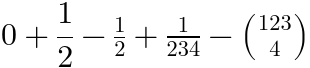The <mfrac> element sets displaystyle to false, or if it was already false increments scriptlevel by 1, within its children. It sets math-shift to compact within its second child. To avoid visual confusion between the fraction bar and another adjacent items (e.g. minus sign or another fraction's bar), a default 1-pixel space is added around the element. The user agent stylesheet must contain the following rules:

If the <mfrac> element has less or more than two in-flow children, its layout algorithm is the same as the [^mrow^] element. Otherwise, the first in-flow child is called numerator, the second in-flow child is called denominator and the layout algorithm is explained below.

In practice, an <mfrac> element has two children that are in-flow. Hence the CSS rules basically perform scriptlevel, displaystyle and math-shift changes for the numerator and denominator.
##### Fraction with nonzero line thickness

If the fraction line thickness is nonzero, the <mfrac> element is laid out as shown on . The fraction bar must only be painted if the visibility of the <mfrac> element is visible. In that case, the fraction bar must be painted with the color of the <mfrac> element.

The min-content inline size (respectively max-content inline size) of content is the maximum between the min-content inline size (respectively max-content inline size) of the numerator's margin box and the min-content inline size (respectively max-content inline size) of the denominator's margin box.

If there is an inline stretch size constraint or a block stretch size constraint then the numerator is also laid out with the same stretch size constraint, otherwise it is laid out without any stretch size constraint. The denominator is always laid out without any stretch size constraint.

The inline size of the math content is the maximum between the inline size of the numerator's margin box and the inline size of the denominator's margin box.

NumeratorShift is the maximum between:

DenominatorShift is the maximum between:

The line-ascent of the math content is the maximum between:

The line-descent of the math content is the maximum between:

The inline offset of the numerator (respectively denominator) is half the inline size of the math content − half the inline size of the numerator's margin box (respectively denominator's margin box).

The alphabetic baseline of the numerator (respectively denominator) is shifted away from the alphabetic baseline by a distance of NumeratorShift (respectively DenominatorShift) towards the line-over (respectively line-under).

The math content box is placed within the content box so that their block-start edges are aligned and the middles of these edges are at the same position.

The inline size of the fraction bar is the inline size of the content box and its inline-start edge is the aligned with the one the content box. The center of the fraction bar is shifted away from the alphabetic baseline of the math content box by a distance of AxisHeight towards the line-over. Its block size is the fraction line thickness.

##### Fraction with zero line thickness

If the fraction line thickness is zero, the <mfrac> element is instead laid out as shown on .

The min-content inline size, max-content inline size and inline size of the math content are calculated the same as in .

If there is an inline stretch size constraint or a block stretch size constraint then the numerator is also laid out with the same stretch size constraint and otherwise it is laid out without any stretch size constraint. The denominator is always laid out without any stretch size constraint.

If the math-style is compact then TopShift and BottomShift are respectively set to StackTopShiftUp and StackBottomShiftDown. Otherwise math-style is normal and they are respectively set to StackTopDisplayStyleShiftUp and StackBottomDisplayStyleShiftDown.

The Gap is defined to be (BottomShift − the ink line-ascent of the denominator's margin box) + (TopShift − the ink line-descent of the numerator's margin box). If math-style is compact then GapMin is StackGapMin, otherwise math-style is normal and it is StackDisplayStyleGapMin. If Δ = GapMinGap is positive then TopShift and BottomShift are respectively increased by Δ/2 and Δ − Δ/2.

The line-ascent of the math content is the maximum between:

The line-descent of the math content is the maximum between:

The inline offsets of the numerator and denominator are calculated the same as in .

The alphabetic baseline of the numerator (respectively denominator) is shifted away from the alphabetic baseline by a distance of TopShift (respectively − BottomShift) towards the line-over (respectively line-under).

The math content box is placed within the content box so that their block-start edges are aligned and the middles of these edges are at the same position.

#### Radicals <msqrt>, <mroot>

The radical elements construct an expression with a root symbol √ with a line over the content. The msqrt element is used for square roots, while the mroot element is used to draw radicals with indices, e.g. a cube root.

The <msqrt> and <mroot> elements accept the attributes described in .

The following example contains a square root written with [^msqrt^] and a cube root written with [^mroot^]. Note that [^msqrt^] has several children and the square root applies to all of them. [^mroot^] has exactly two children: it is a root of index the second child (the number 3), applied to the first child (the square root). Also note these elements only change the font-size within the [^mroot^] index, but it is scaled down more than within the numerator and denumerator of the fraction.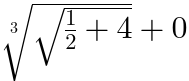The <msqrt> and <mroot> elements sets math-shift to compact. The <mroot> element increments scriptlevel by 2, and sets displaystyle to "false" in all but its first child. The user agent stylesheet must contain the following rule in order to implement that behavior:

If the <msqrt> or <mroot> element do not have their computed [^math/display^] property equal to block math or inline math then they are laid out according to the CSS specification where the corresponding value is described. Otherwise, the layout below is performed.

If the <mroot> has less or more than two in-flow children, its layout algorithm is the same as the [^mrow^] element. Otherwise, the first in-flow child is called mroot base and the second in-flow child is called mroot index and its layout algorithm is explained below.

In practice, an <mroot> element has two children that are in-flow. Hence the CSS rules basically perform scriptlevel and displaystyle changes for the index.

The <msqrt> element generates an anonymous <mrow> box called the msqrt base.

The radical symbol must only be painted if the visibility of the <msqrt> or <mroot> element is visible. In that case, the radical symbol must be painted with the color of that element.

The radical glyph is the glyph obtained for the character U+221A SQUARE ROOT.

The radical gap is given by RadicalVerticalGap if the math-style is compact and RadicalDisplayStyleVerticalGap if the math-style is normal.

The radical target size for the stretchy radical glyph is the sum of RadicalRuleThickness, radical gap and the ink height of the base.

The box metrics of the radical glyph and painting of the surd are given by the algorithm to shape a stretchy glyph to block dimension the target size for the radical glyph.

##### Square root

The <msqrt> element is laid out as shown on .

The min-content inline size (respectively max-content inline size) of the math content is the sum of the preferred inline size of a glyph stretched along the block axis for the radical glyph and of the min-content inline size (respectively max-content inline size) of the msqrt base's margin box.

The inline size of the math content is the sum of the advance width of the box metrics of the radical glyph and of the inline size of the msqrt base's margin's box.

The line-ascent of the math content is the maximum between:

The line-descent of the math content is the maximum between:

The inline size of the overbar is the inline size of the msqrt base's margin's box. The inline offsets of the msqrt base and overbar are also the same and equal to the width of the box metrics of the radical glyph.

The alphabetic baseline of the msqrt base is aligned with the alphabetic baseline. The block size of the overbar is RadicalRuleThickness. Its vertical center is shifted away from the alphabetic baseline by a distance towards the line-over equal to the line-ascent of the math content, minus the RadicalExtraAscender, minus half the RadicalRuleThickness.

Finally, the painting of the surd is performed:

##### Root with index

The <mroot> element is laid out as shown on . The mroot index is first ignored and the mroot base and radical glyph are laid out as shown on figure using the same algorithm as in in order to produce a margin box B (represented in green).

The min-content inline size (respectively max-content inline size) of the math content is the sum of max(0, RadicalKernBeforeDegree), the mroot index's min-content inline size (respectively max-content inline size) of the mroot index's margin box, max(−min-content inline size, RadicalKernAfterDegree) (respectively max(−max-content inline size, RadicalKernAfterDegree)) and of the min-content inline size (respectively max-content inline size) of B.

The inline size of the math content is the sum of AdjustedRadicalKernBeforeDegree, the inline size of the index's margin box, AdjustedRadicalKernAfterDegree and of the inline size of B.

The line-ascent of the math content is the maximum between:

The line-descent of the math content is the maximum between:

The inline offset of the index is AdjustedRadicalKernBeforeDegree. The inline-offset of the mroot base is the same + the inline size of the index's margin box.

The alphabetic baseline of B is aligned with the alphabetic baseline. The alphabetic baseline of the index is shifted away from the line-under edge by a distance of RadicalDegreeBottomRaisePercent × the block size of B + the line-descent of the index's margin box.

In general, the kerning before the root index is positive while the kerning after it is negative, which means that the root element will have some inline-start space and that the root index will overlap the surd.

#### Style Change <mstyle>

Historically, the mstyle element was introduced to make style changes that affect the rendering of its contents.

The <mstyle> element accepts the attributes described in . Its layout algorithm is the same as the [^mrow^] element.

<mstyle> is implemented for compatibility with full MathML. Authors whose only target is MathML Core are encouraged to use CSS for styling.

In the following example, [^mstyle^] is used to set the scriptlevel and displaystyle. Observe this is respectively affecting the font-size and placement of subscripts of their descendants. In MathML Core, one could just have used [^mrow^] elements instead.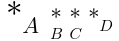#### Error Message <merror>

The merror element displays its contents as an ”error message”. The intent of this element is to provide a standard way for programs that generate MathML from other input to report syntax errors in their input.

In the following example, [^merror^] is used to indicate a parsing error for some LaTeX-like input: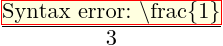The <merror> element accepts the attributes described in . Its layout algorithm is the same as the [^mrow^] element. The user agent stylesheet must contain the following rule in order to visually highlight the error message:

#### Adjust Space Around Content <mpadded>

The mpadded element renders the same as its in-flow child content, but with the size and relative positioning point of its content modified according to <mpadded>’s attributes.

The <mpadded> element accepts the attributes described in as well as the following attributes:

The width, height, depth, lspace and voffset if present, must have a value that is a valid <length-percentage>.

In the following example, [^mpadded^] is used to tweak spacing around a fraction (a blue background is used to visualize it). Without attributes, it behaves like an [^mrow^] but the attributes allow to specify the size of the box (width, height, depth) and position of the fraction within that box (lspace and voffset).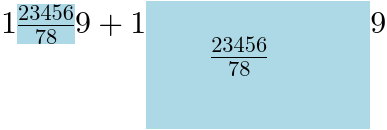##### Inner box and requested parameters

The [^mpadded^] element generates an anonymous <mrow> box called the mpadded inner box with parameters called inner inline size, inner line-ascent and inner line-descent.

The requested <mpadded> parameters are determined as follows:

• The requested width is the resolved value of the width property. If the width attribute is present, valid and not a percentage then that attribute is used as a presentational hint setting the element's width property to the corresponding value.
• If the height attribute is absent, invalid or a percentage then the requested height is the inner line-ascent. Otherwise the requested height is the resolved value of the height attribute, clamping negative values to 0.
• If the depth attribute is absent, invalid or a percentage then the requested depth is the inner line-ascent. Otherwise the requested depth is the resolved value of the depth attribute, clamping negative values to 0.
• If the lspace attribute is absent, invalid or a percentage then the requested lspace is 0. Otherwise the requested lspace is the resolved value of the lspace attribute, clamping negative values to 0.
• If the voffset attribute is absent, invalid or a percentage then the requested voffset is 0. Otherwise the requested voffset is the resolved value of the voffset attribute.
Negative voffset values are not clamped to 0.
##### Layout of <mpadded>

If the <mpadded> element does not have its computed [^math/display^] property equal to block math or inline math then it is laid out according to the CSS specification where the corresponding value is described. Otherwise, it is laid out as shown on .

The min-content inline size (respectively max-content inline size) of the math content is the requested width calculated in but using the min-content inline size (respectively max-content inline size) of the mpadded inner box instead of the "inner inline size".

The inline size of the math content is the requested width calculated in .

The line-ascent of the math content is the requested height. The line-descent of the math content is the requested depth.

The mpadded inner box is placed so that its alphabetic baseline is shifted away from the alphabetic baseline by the requested voffset towards the line-over.

#### Making Sub-Expressions Invisible <mphantom>

Historically, the mphantom element was introduced to render its content invisibly, but with the same metrics size and other dimensions, including alphabetic baseline position that its contents would have if they were rendered normally.

In the following example, [^mphantom^] is used to ensure alignment of corresponding parts of the numerator and denominator of a fraction: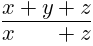The <mphantom> element accepts the attributes described in . Its layout algorithm is the same as the [^mrow^] element. The user agent stylesheet must contain the following rule in order to hide the content:

<mphantom> is implemented for compatibility with full MathML. Authors whose only target is MathML Core are encouraged to use CSS for styling.

### Script and Limit Schemata

The elements described in this section position one or more scripts around a base. Attaching various kinds of scripts and embellishments to symbols is a very common notational device in mathematics. For purely visual layout, a single general-purpose element could suffice for positioning scripts and embellishments in any of the traditional script locations around a given base. However, in order to capture the abstract structure of common notation better, MathML provides several more specialized scripting elements.

In addition to sub-/superscript elements, MathML has overscript and underscript elements that place scripts above and below the base. These elements can be used to place limits on large operators, or for placing accents and lines above or below the base.

#### Subscripts and Superscripts <msub>, <msup>, <msubsup>

The msub, msup and msubsup elements are used to attach subscript and superscript to a MathML expression. They accept the attributes described in .

The following example shows basic use of subscripts and superscripts. The font-size is automatically scaled down within the scripts.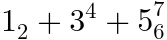If the <msub>, <msup> or <msubsup> elements do not have their computed [^math/display^] property equal to block math or inline math then they are laid out according to the CSS specification where the corresponding value is described. Otherwise, the layout below is performed.

##### Children of <msub>, <msup>, <msubsup>

If the <msub> element has less or more than two in-flow children, its layout algorithm is the same as the [^mrow^] element. Otherwise, the first in-flow child is called the msub base, the second in-flow child is called the msub subscript and the layout algorithm is explained in .

If the <msup> element has less or more than two in-flow children, its layout algorithm is the same as the [^mrow^] element. Otherwise, the first in-flow child is called the msup base, the second in-flow child is called the msup superscript and the layout algorithm is explained in .

If the <msubsup> element has less or more than three in-flow children, its layout algorithm is the same as the [^mrow^] element. Otherwise, the first in-flow child is called the msubsup base, the second in-flow child is called the msubsup subscript, its third in-flow child is called the msubsup superscript and the layout algorithm is explained in .

##### Base with subscript

The <msub> element is laid out as shown on . LargeOpItalicCorrection is the italic correction of the msub base if it is an embellished operator with the [=embellished operator/largeop=] property and 0 otherwise.

The min-content inline size (respectively max-content inline size) of the math content is the min-content inline size (respectively max-content inline size) of the msub base's margin boxLargeOpItalicCorrection + min-content inline size (respectively max-content inline size) of the msub subscript's margin box + SpaceAfterScript.

If there is an inline stretch size constraint or a block stretch size constraint then the msub base is also laid out with the same stretch size constraint and otherwise it is laid out without any stretch size constraint. The scripts are always laid out without any stretch size constraint.

The inline size of the math content is the inline size of the msub base's margin boxLargeOpItalicCorrection + the inline size of the msub subscript's margin box + SpaceAfterScript.

SubShift is the maximum between:

The line-ascent of the math content is the maximum between:

The line-descent of the math content is the maximum between:

The inline offset of the msub base is 0 and the inline offset of the msub subscript is the inline size of the msub base's margin boxLargeOpItalicCorrection.

The msub base is placed so that its alphabetic baseline matches the alphabetic baseline. The msub subscript is placed so that its alphabetic baseline is shifted away from the alphabetic baseline by SubShift towards the line-under.

##### Base with superscript

The <msup> element is laid out as shown on . ItalicCorrection is the italic correction of the msup base if it is not an embellished operator with the [=embellished operator/largeop=] property and 0 otherwise.

The min-content inline size (respectively max-content inline size) of the math content is the min-content inline size (respectively max-content inline size) of the msup base's margin box + ItalicCorrection + the min-content inline size (respectively max-content inline size) of the msup superscript's margin box + SpaceAfterScript.

If there is an inline stretch size constraint or a block stretch size constraint then the msup base is also laid out with the same stretch size constraint and otherwise it is laid out without any stretch size constraint. The scripts are always laid out without any stretch size constraint.

The inline size of the math content is the inline size of the msup base's margin box + ItalicCorrection + the inline size of the msup superscript's margin box + SpaceAfterScript.

SuperShift is the maximum between:

The line-ascent of the math content is the maximum between:

The line-descent of the math content is the maximum between:

The inline offset of the msup base is 0 and the inline offset of msup superscript is the inline size of the msup base's margin box + ItalicCorrection.

The msup base is placed so that its alphabetic baseline matches the alphabetic baseline. The msup superscript is placed so that its alphabetic baseline is shifted away from the alphabetic baseline by SuperShift towards the line-over.

##### Base with subscript and superscript

The <msubsup> element is laid out as shown on . LargeOpItalicCorrection and SubShift are set as in . ItalicCorrection and SuperShift are set as in .

The min-content inline size (respectively max-content inline size and inline size) of the math content is the maximum between the min-content inline size (respectively max-content inline size and inline size) of the math content calculated in and .

If there is an inline stretch size constraint or a block stretch size constraint then the msubsup base is also laid out with the same stretch size constraint and otherwise it is laid out without any stretch size constraint. The scripts are always laid out without any stretch size constraint.

If there is an inline stretch size constraint or a block stretch size constraint then the msubsup base is also laid out with the same stretch size constraint and otherwise it is laid out without any stretch size constraint. The scripts are always laid out without any stretch size constraint.

SubSuperGap is the gap between the two scripts along the block axis and is defined by (SubShift − the ink line-ascent of the msubsup subscript's margin box) + (SuperShift − the ink line-descent of the msubsup superscript's margin box). If SubSuperGap is not at least SubSuperscriptGapMin then the following steps are performed to ensure that the condition holds:

1. Let Δ be SuperscriptBottomMaxWithSubscript − (SuperShift − the ink line-descent of the msubsup superscript's margin box). If Δ > 0 then set Δ to the minimum between Δ set SubSuperscriptGapMinSubSuperGap and increase SuperShift (and so SubSuperGap too) by Δ.
2. Let Δ be SubSuperscriptGapMinSubSuperGap. If Δ > 0 then increase SubscriptShift (and so SubSuperGap too) by Δ.

The ink line-ascent (respectively line-ascent, ink line-descent, line-descent) of the math content is set to the maximum of the ink line-ascent (respectively line-ascent, ink line-descent, line-descent) of the math content calculated in and but using the adjusted values SubShift and SuperShift above.

The inline offset and block offset of the msubsup base and scripts are performed the same as described in and .

Even when the msubsup subscript (respectively msubsup superscript) is an empty box, <msubsup> does not generally render the same as (respectively ) because of the additional constraint on SubSuperGap. Moreover, positioning the empty msubsup subscript (respectively msubsup superscript) may also change the total size.

In order to keep the algorithm simple, no attempt is made to handle empty scripts in a special way.

#### Underscripts and Overscripts <munder>, <mover>, <munderover>

The munder, mover and munderover elements are used to attach accents or limits placed under or over a MathML expression.

The <munderover> element accepts the attribute described in as well as the following attributes:

• [^munderover/accent^]
• [^munderover/accentunder^]

Similarly, the <mover> element (respectively <munder> element) accepts the attribute described in as well as the [^mover/accent^] attribute (respectively the [^mover/accentunder^] attribute).

accent, accentunder attributes, if present, must have values that are booleans. If these attributes are absent or invalid, they are treated as equal to false. User agents must implement them as described in .

The following example shows basic use of under- and overscripts. The font-size is automatically scaled down within the scripts, unless they are meant to be accents.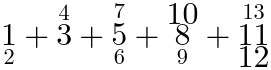If the <munder>, <mover> or <munderover> elements do not have their computed [^math/display^] property equal to block math or inline math then they are laid out according to the CSS specification where the corresponding value is described. Otherwise, the layout below is performed.

##### Children of <munder>, <mover>, <munderover>

If the <munder> element has less or more than two in-flow children, its layout algorithm is the same as the [^mrow^] element. Otherwise, the first in-flow child is called the munder base and the second in-flow child is called the munder underscript.

If the <mover> element has less or more than two in-flow children, its layout algorithm is the same as the [^mrow^] element. Otherwise, the first in-flow child is called the mover base and the second in-flow child is called the mover overscript.

If the <munderover> element has less or more than three in-flow children, its layout algorithm is the same as the [^mrow^] element. Otherwise, the first in-flow child is called the munderover base, the second in-flow child is called the munderover underscript and its third in-flow child is called the munderover overscript.

If the <munder>, <mover> or <munderover> elements have a computed math-style property equal to compact and their base is an embellished operator with the [=embellished operator/movablelimits=] property, then their layout algorithms are respectively the same as the ones described for <msub>, <msup> and <msubsup> in , and .

Otherwise, the <munder>, <mover> and <munderover> layout algorithms are respectively described in , and .

#### Algorithm for stretching operators along the inline axis

The algorithm for stretching operators along the inline axis is as follows.

1. If there is an inline stretch size constraint or block stretch size constraint then the element being laid out is an embellished operator. Lay out the base with the same stretch size constraint.
2. Split the list of in-flow children that have not been laid out yet into a first list LToStretch containing embellished operators with a [=embellished operator/stretchy=] property and inline [=embellished operator/stretch axis=]; and a second list LNotToStretch.
3. Perform layout without any stretch size constraint on all the items of LNotToStretch. If LToStretch is empty then stop. If LNotToStretch is empty, perform layout with stretch size constraint 0 on all the items of LToStretch.
4. Calculate the target size T to the maximum inline size of the margin boxes of child boxes that have been laid out in the previous step.
5. Lay out or relayout all the elements of LToStretch with inline stretch size constraint T.

#### Base with underscript

The <munder> element is laid out as shown on . LargeOpItalicCorrection is the italic correction of the munder base if it is an embellished operator with the [=embellished operator/largeop=] property and 0 otherwise.

The min-content inline size (respectively max-content inline size) of the math content are calculated like the inline size of the math content below but replacing the inline sizes of the munder base's margin box and munder underscript's margin box with the min-content inline size (respectively max-content inline size) of the munder base's margin box and munder underscript's margin box.

The in-flow children are laid out using the algorithm for stretching operators along the inline axis.

The inline size of the math content is calculated by determining the absolute difference between:

If m is the minimum calculated in the second item above then the inline offset of the munder base is −m − half the inline size of the base's margin box. The inline offset of the munder underscript is −m − half the inline size of the munder underscript's margin box − half LargeOpItalicCorrection.

Parameters UnderShift and UnderExtraDescender are determined by considering three cases in the following order:

1. The munder base is an embellished operator with the [=embellished operator/largeop=] property. UnderShift is the maximum of

UnderExtraDescender is 0.

2. The munder base is an embellished operator with the [=embellished operator/stretchy=] property and [=embellished operator/stretch axis=] inline. UnderShift is the maximum of:

UnderExtraDescender is 0.
3. Otherwise, UnderShift is equal to UnderbarVerticalGap if the [^munder/accentunder^] attribute is not an ASCII case-insensitive match to true and to zero otherwise. UnderExtraAscender is UnderbarExtraDescender.

The line-ascent of the math content is the maximum between:

The line-descent of the math content is the maximum between:

The alphabetic baseline of the munder base is aligned with the alphabetic baseline. The alphabetic baseline of the munder underscript is shifted away from the alphabetic baseline and towards the line-under by a distance equal to the ink line-descent of the munder base's margin box + UnderShift.

The math content box is placed within the content box so that their block-start edges are aligned and the middles of these edges are at the same position.

#### Base with overscript

The <mover> element is laid out as shown on . LargeOpItalicCorrection is the italic correction of the mover base if it is an embellished operator with the [=embellished operator/largeop=] property and 0 otherwise.

The min-content inline size (respectively max-content inline size) of the math content are calculated like the inline size of the math content below but replacing the inline sizes of the mover base's margin box and mover overscript's margin box with the min-content inline size (respectively max-content inline size) of the mover base's margin box and mover overscript's margin box.

The in-flow children are laid out using the algorithm for stretching operators along the inline axis.

The TopAccentAttachment is the top accent attachment of the mover overscript or half the inline size of the mover overscript's margin box if it is undefined.

The inline size of the math content is calculated by applying the algorithm for stretching operators along the inline axis for layout and determining the absolute difference between:

If m is the minimum calculated in the second item above then the inline offset of the mover base is −m − half the inline size of the base's margin. The inline offset of the mover overscript is −m − half the inline size of the mover overscript's margin box + half LargeOpItalicCorrection.

Parameters OverShift and OverExtraDescender are determined by considering three cases in the following order:

1. The mover base is an embellished operator with the [=embellished operator/largeop=] property. OverShift is the maximum of

OverExtraAscender is 0.

2. The mover base is an embellished operator with the [=embellished operator/stretchy=] property and [=embellished operator/stretch axis=] inline. OverShift is the maximum of:

OverExtraDescender is 0.
3. Otherwise, OverShift is equal to

1. OverbarVerticalGap if the [^mover/accent^] attribute is not an ASCII case-insensitive match to true.
2. Or AccentBaseHeight minus the line-ascent of the mover base's margin box if this difference is nonnegative.
3. Or 0 otherwise.

OverExtraAscender is OverbarExtraAscender.

For accent overscripts and bases with line-ascents that are at most AccentBaseHeight, the rule from [[OPEN-FONT-FORMAT]] [[?TEXBOOK]] is actually to align the alphabetic baselines of the overscripts and of the bases. This assumes that accent glyphs are designed in such a way that their ink bottoms are more or less AccentBaseHeight above their alphabetic baselines. Hence, the previous rule will guarantee that all the overscript bottoms are aligned while still avoiding collision with the bases. However, MathML can have arbitrary accent overscripts, so a more general and simpler rule is provided above: Ensure that the bottom of overscript is at least AccentBaseHeight above the alphabetic baseline of the base.

The line-ascent of the math content is the maximum between:

The line-descent of the math content is the maximum between:

The alphabetic baseline of the mover base is aligned with the alphabetic baseline. The alphabetic baseline of the mover overscript is shifted away from the alphabetic baseline and towards the line-over by a distance equal to the ink line-ascent of the base + OverShift.

The math content box is placed within the content box so that their block-start edges are aligned and the middles of these edges are at the same position.

#### Base with underscript and overscript

The general layout of <munderover> is shown on . The LargeOpItalicCorrection, UnderShift, UnderExtraDescender, OverShift, OverExtraDescender parameters are calculated the same as in and .

The min-content inline size, max-content inline size and inline size of the math content are calculated as an absolute difference between a maximum inline offset and minimum inline offset. These extrema are calculated by taking the extremum value of the corresponding extrema calculated in and . The inline offsets of the munderover base, munderover underscript and munderover overscript are calculated as in these sections but using the new minimum m (minimum of the corresponding minima).

Like in these sections, the in-flow children are laid out using the algorithm for stretching operators along the inline axis.

The line-ascent and line-descent of the math content are also calculated by taking the extremum value of the extrema calculated in and .

Finally, the alphabetic baselines of the munderover base, munderover underscript and munderover overscript are calculated as in sections and .

The math content box is placed within the content box so that their block-start edges are aligned and the middles of these edges are at the same position.

When the underscript (respectively overscript) is an empty box, the base and overscript (respectively underscript) are laid out similarly to (respectively ) but the position of the empty underscript (respectively overscript) may add extra space. In order to keep the algorithm simple, no attempt is made to handle empty scripts in a special way.

#### Prescripts and Tensor Indices <mmultiscripts>

Presubscripts and tensor notations are represented by the mmultiscripts element. The mprescripts element is used as a separator between the postscripts and prescripts. These two elements accept the attributes described in .

The following example shows basic use of prescripts and postscripts, involving a [^mprescripts^]. Empty [^mrow^] elements are used at positions where no scripts are rendered. The font-size is automatically scaled down within the scripts.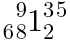If the <mmultiscripts> or <mprescripts> elements do not have their computed [^math/display^] property equal to block math or inline math then they are laid out according to the CSS specification where the corresponding value is described. Otherwise, the layout below is performed.

The <mprescripts> element is laid out as an [^mrow^] element.

A valid <mmultiscripts> element contains the following in-flow children:

• A first in-flow child, called the mmultiscripts base, that is not an [^mprescripts^] element.
• Followed by an even number of in-flow children called mmultiscripts postscripts, none of them being a [^mprescripts^] element. These scripts form a (possibly empty) list subscript, superscript, subscript, superscript, subscript, superscript, etc. Each consecutive couple of children subscript, superscript is called a subscript/superscript pair.
• Optionally followed by an [^mprescripts^] element and an even number of in-flow children called mmultiscripts prescripts, none of them being a [^mprescripts^] element. These scripts form a (possibly empty) list of subscript/superscript pair.

If an <mmultiscripts> element is not valid then it is laid out the same as the [^mrow^] element. Otherwise the layout algorithm is performed as in .

##### Base with prescripts and postscripts

The <mmultiscripts> element is laid out as shown on . For each subscript/superscript pair of mmultiscripts postscripts, the ItalicCorrection LargeOpItalicCorrection are defined as in and .

The min-content inline size (respectively max-content inline size) of the math content is calculated the same as the inline size of the math content below, but replacing "inline size" with "min-content inline size" (respectively "max-content inline size") for the mmultiscripts base's margin box and scripts' margin boxes.

If there is an inline stretch size constraint or a block stretch size constraint the mmultiscripts base is also laid out with the same stretch size constraint. Otherwise it is laid out without any stretch size constraint. The other elements are always laid out without any stretch size constraint.

The inline size of the math content is calculated with the following algorithm:

1. Set inline-offset to 0.
2. For each subscript/superscript pair of mmultiscripts prescripts, increment inline-offset by SpaceAfterScript + the maximum of

3. Increment inline-offset by the inline size of the mmultiscripts base's margin box and set inline-size to inline-offset.
4. For each subscript/superscript pair of mmultiscripts postscripts, modify inline-size to be at least:

Increment inline-offset to the maximum of:

Increment inline-offset by SpaceAfterScript.

5. Return inline-size.

SubShift (respectively SuperShift) is calculated by taking the maximum of all subshifts (respectively supershifts) of each subscript/superscript pair as described in .

The line-ascent of the math content is calculated by taking the maximum of all the line-ascent of each subscript/superscript pair as described in but using the SubShift and SuperShift values calculated above.

The line-descent of the math content is calculated by taking the maximum of all the line-descent of each subscript/superscript pair as described in but using the SubShift and SuperShift values calculated above.

Finally, the placement of the in-flow children is performed using the following algorithm:

1. Set inline-offset to 0.
1. Increment inline-offset by SpaceAfterScript.
2. Set pair-inline-size to the maximum of
3. Place the subscript at inline-start position inline-offset + pair-inline-size − the inline size of the subscript's margin box.
4. Place the superscript at inline-start position inline-offset + pair-inline-size − the inline size of the superscript's margin box.
5. Place the subscript (respectively superscript) so its alphabetic baseline is shifted away from the alphabetic baseline by SubShift (respectively SuperShift) towards the line-under (respectively line-over).
6. Increment inline-offset by pair-inline-size.
2. Place the mmultiscripts base and <mprescripts> boxes at inline offsets inline-offset and with their alphabetic baselines aligned with the alphabetic baseline.
1. Set pair-inline-size to the maximum of
2. Place the subscript at inline-start position inline-offsetLargeOpItalicCorrection.
3. Place the superscript at inline-start position inline-offset + ItalicCorrection.
4. Place the subscript (superscript) so its alphabetic baseline is shifted away from the alphabetic baseline by SubShift (respectively SuperShift) towards the line-under (respectively line-over).
5. Increment inline-offset by pair-inline-size.
6. Increment inline-offset by SpaceAfterScript.

An <mmultiscripts> with only one subscript/superscript pair of mmultiscripts postscripts is laid out the same as a <msubsup> with the same in-flow children. However, as noticed for <msubsup>, if additionally the subscript (respectively superscript) is an empty box then it is not necessarily laid out the same as an <msub> (respectively <msup>) element. In order to keep the algorithm simple, no attempt is made to handle empty scripts in a special way.

#### Displaystyle, scriptlevel and math-shift in scripts

For all scripted elements, the rule of thumb is to set displaystyle to false and to increment scriptlevel in all child elements but the first one. However, an [^mover^] (respectively [^munderover^]) element with an [^mover/accent^] attribute that is an ASCII case-insensitive match to true does not increment scriptlevel within its second child (respectively third child). Similarly, [^mover^] and [^munderover^] elements with an [^mover/accentunder^] attribute that is an ASCII case-insensitive match to true do not increment scriptlevel within their second child.

<mmultiscripts> sets math-shift to compact on its children at even position if they are before an [^mprescripts^], and on those at odd position if they are after an [^mprescripts^]. The <msub> and <msubsup> elements set math-shift to compact on their second child. [^mover^] and [^munderover^] elements with an [^mover/accent^] attribute that is an ASCII case-insensitive match to true also set math-shift to compact within their first child.

The must contain the following style in order to implement this behavior:

In practice, all the children of the MathML elements described in this section are in-flow and the <mprescripts> is empty. Hence the CSS rules essentially perform automatic displaystyle and scriptlevel changes for the scripts; and math-shift changes for subscripts and sometimes the base.

### Tabular Math

Matrices, arrays and other table-like mathematical notation are marked up using [^mtable^] [^mtr^] [^mtd^] elements. These elements are similar to the [^table^], [^tr^] and [^td^] elements of [[HTML]].

The following example shows how tabular layout allows to write a matrix. Note that it is vertically centered with the fraction bar and the middle of the equal sign.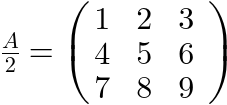#### Table or Matrix <mtable>

The mtable is laid out as an inline-table and sets displaystyle to false. The user agent stylesheet must contain the following rules in order to implement these properties:

The mtable element is as a CSS table and the min-content inline size, max-content inline size, inline size, block size, first baseline set and last baseline set sets are determined accordingly. The center of the table is aligned with the math axis.

The <mtable> accepts the attributes described in .

#### Row in Table or Matrix <mtr>

The mtr is laid out as table-row. The user agent stylesheet must contain the following rules in order to implement that behavior:

The <mtr> accepts the attributes described in .

#### Entry in Table or Matrix <mtd>

The mtd is laid out as a table-cell with content centered in the cell and a default padding. The user agent stylesheet must contain the following rules:

The <mtd> accepts the attributes described in as well as the following attributes:

The colspan (respectively rowspan) attribute has the same syntax and semantics as the [^td/colspan^] (respectively [^td/rowspan^]) attribute on the <td> element from [[HTML]]. In particular, the parsing of these attributes is handled as described in the algorithm for processing rows.

The name of the column spanning attribute in [[MathML3]] and earlier versions was columnspan. For consistency with [[HTML]], this specification uses colspan instead.

The <mtd> element generates an anonymous <mrow> box.

### Enlivening Expressions

Historically, the maction element provides a mechanism for binding actions to expressions.

The <maction> element accepts the attributes described in as well as the following attributes:

• [^maction/actiontype^]
• [^maction/selection^]

This specification does not define any observable behavior that is specific to the actiontype and selection attributes.

The following example shows the "toggle" action type from [[MathML3]] where the renderer alternately displays the selected subexpression, starting from "one third" and cycling through them when there is a click on the selected subexpression ("one quarter", "one half", "one third", etc). This is not part of MathML Core but can be implemented using JavaScript and CSS polyfills. The default behavior is just to render the first child.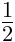The layout algorithm of the <maction> element is the same as the <mrow> element. The user agent stylesheet must contain the following rules in order to hide all but its first child element, which is the default behavior for the legacy actiontype values:

<maction> is implemented for compatibility with full MathML. Authors whose only target is MathML Core are encouraged to use other HTML, CSS and JavaScript mechanisms to implement custom actions. They may rely on maction attributes defined in [[MathML3]].

### Semantics and Presentation

The semantics element is the container element that associates annotations with a MathML expression. Typically, the <semantics> element has as its first child element a MathML expression to be annotated while subsequent child elements represent text annotations within an annotation element, or more complex markup annotations within an annotation-xml element.

The following example shows how the fraction "one half" can be annotated with a textual annotation (LaTeX) or an XML annotation (content MathML). These annotations are not intended to be rendered by the user agent.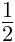The <semantics> element accepts the attributes described in . Its layout algorithm is the same as the [^mrow^] element. The user agent stylesheet must contain the following rule in order to only render the annotated MathML expression:

The <annotation-xml> and <annotation> element accepts the attributes described in as well as the following attribute:

• [^annotation/encoding^]

This specification does not define any observable behavior that is specific to the encoding attribute.

The layout algorithm of the <annotation-xml> and <annotation> element is the same as the [^mtext^] element.

Authors can use the [^annotation/encoding^] attribute to distinguish annotations for HTML integration point, clipboard copy, alternative rendering, etc. In particular, CSS can be used to render alternative annotations, e.g.
            /* Hide the annotated child. */
semantics > :first-child { display: none; }
/* Show all text annotations. */
semantics > annotation { display: inline; }
/* Show all HTML annotations. */
semantics > annotation-xml[encoding="text/html" i],
semantics > annotation-xml[encoding="application/xhtml+xml" i] {
display: inline-block;
}


## CSS Extensions for Math Layout

### The display: block math and display: inline math value

The display property from is extended with a new inner display type:

Name: display || [ | math ]

For elements that are not MathML elements, if the specified value of display is inline math or block math then the computed value is block flow and inline flow respectively. For the [^mtable^] element the computed value is block table and inline table respectively. For the [^mtr^] element, the computed value is table-row. For the [^mtd^] element, the computed value is table-cell.

MathML elements with a computed display value equal to block math or inline math control box generation and layout according to their tag name, as described in the relevant sections. Unknown MathML elements behave the same as the [^mrow^] element.

The display: block math and display: inline math values provide a default layout for MathML elements while at the same time allowing to override it with either native display values or custom values. This allows authors or polyfills to define their own custom notations to tweak or extend MathML Core.

In the following example, the default layout of the MathML [^mrow^] element is overridden to render its content as a grid.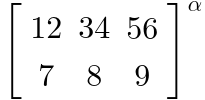### New text-transform value

The text-transform property from is extended with a new value:

Name: text-transform math-auto

On text nodes containing a single character, if the computed value is math-auto then the transformed text is obtained by performing conversion of each character according to the italic table.

A common style convention is to render identifiers with multiple letters (e.g. the function name "exp") with normal style and identifiers with a single letter (e.g. the variable "n") with italic style. The math-auto property is intended to implement this default behavior, which can be overridden by authors if necessary. Note that mathematical fonts are designed with a special kind of italic glyphs located at the Unicode positions of , which differ from the shaping obtained via italic font style. Compare this mathematical formula rendered with the Latin Modern Math font using font-style: italic (left) and text-transform: math-auto (right):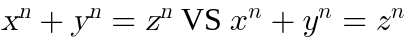### The math-style property

Name: math-style normal | compact normal All elements yes n/a specified keyword n/a not animatable visual

When math-style is compact, the math layout on descendants tries to minimize the logical height by applying the following rules:

• The font-size is scaled down when its specified value is math and the computed value of math-depth is auto-add (default for [^mfrac^]) as described in .
• Operators with the [=embellished operator/largeop=] property do not follow rules from to make them bigger.
• Under-/overscripts attached to an operator with the [=embellished operator/movablelimits=] property are actually drawn as sub-/superscripts as described in .
• Smaller vertical gaps and shifts from the OpenType MATH table are used for fractions and radicals, as described in , and .

The following example shows a mathematical formula rendered with its [^math^] root styled with math-style: compact (left) and math-style: normal (right). In the former case, the font-size is automatically scaled down within the fractions and the summation limits are rendered as subscript and superscript of the ∑. In the latter case, the ∑ is drawn bigger than normal text and vertical gaps within fractions (even relative to current font-size) are larger.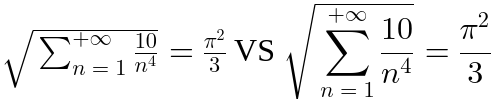These two math-style values typically correspond to mathematical expressions in inline and display mode respectively [[TeXBook]]. A mathematical formula in display mode may automatically switch to inline mode within some subformulas (e.g. scripts, matrix elements, numerators and denominators, etc) and it is sometimes desirable to override this default behavior. The math-style property allows to easily implement these features for MathML in the user agent stylesheet and with the displaystyle attribute; and also exposes them to polyfills.

### The math-shift property

Name: math-shift normal | compact normal All elements yes n/a specified keyword n/a not animatable visual

If the value of math-shift is compact, the math layout on descendants will use the superscriptShiftUpCramped parameter to place superscript. If the value of math-shift is normal, the math will use the superscriptShiftUp parameter instead.

This property is used for positioning superscript during the layout of MathML scripted elements. See § , and .

In the following example, the two "x squared" are rendered with compact math-style and the same font-size. However, the one within the square root is rendered with compact math-shift while the other one is rendered with normal math-shift, leading to subtle different shift of the superscript "2".Per [[TeXBook]], a mathematical formula uses normal style by default but may switch to compact style ("cramped" in TeX's terminology) within some subformulas (e.g. radicals, fraction denominators, etc). The math-shift property allows to easily implement these rules for MathML in the user agent stylesheet. Page authors or developers of polyfills may also benefit from having access to this property to tweak or refine the default implementation.

### The math-depth property

A new math-depth property is introduced to describe a notion of "depth" for each element of a mathematical formula, with respect to the top-level container of that formula. Concretely, this is used to determine the computed value of the font-size property when its specified value is math.

Name: math-depth auto-add | add() | 0 All elements yes n/a an integer, see below n/a not animatable visual

The computed value of the math-depth value is determined as follows:

• If the specified value of math-depth is auto-add and the inherited value of math-style is compact then the computed value of math-depth of the element is its inherited value plus one.
• If the specified value of math-depth is of the form add(<integer>) then the computed value of math-depth of the element is its inherited value plus the specified integer.
• If the specified value of math-depth is of the form <integer> then the computed value of math-depth of the element is the specified integer.
• Otherwise, the computed value of math-depth of the element is the inherited one.

If the specified value font-size is math then the computed value of font-size is obtained by multiplying the inherited value of font-size by a nonzero scale factor calculated by the following procedure:

1. Let A be the inherited math-depth value, B the computed math-depth value, C be 0.71 and S be 1.0
• If A = B then return S.
• If B < A, swap A and B and set InvertScaleFactor to true.
• Otherwise B > A and set InvertScaleFactor to false.
2. Let E be B - A > 0.
3. If the inherited first available font has an OpenType MATH table:
4. Multiply S by CE.
5. Return S if InvertScaleFactor is false and 1/S otherwise.

The following example shows a mathematical formula with normal math-style rendered with the Latin Modern Math font. When entering subexpressions like scripts or fractions, the font-size is automatically scaled down according to the values of MATH table contained in that font. Note that font-size is scaled down when entering the superscripts but even faster when entering a root's prescript. Also it is scaled down when entering the inner fraction but not when entering the outer one, due to automatic change of math-style in fractions.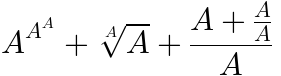These rules from [[TeXBook]] are subtle and it's worth having a separate math-depth mechanism to express and handle them. They can be implemented in MathML using the user agent stylesheet. Page authors or developers of polyfills may also benefit from having access to this property to tweak or refine the default implementation. In particular, the scriptlevel attribute from MathML provides a way to perform math-depth changes.

## OpenType MATH table

This chapter describes features provided by MATH table of an OpenType font [[OPEN-FONT-FORMAT]]. Throughout this chapter, a C-like notation Table.Subtable1[index].Subtable2.Parameter is used to denote OpenType parameters. Such parameters may not be available (e.g. if the font lacks one of the subtable, has an invalid offset, etc) and so fallback options are provided.

It is strongly encouraged to render MathML with a math font with the proper OpenType features. There is no guarantee that the fallback options provided will provide good enough rendering.

OpenType values expressed in design units (perhaps indirectly via a MathValueRecord entry) are scaled to appropriate values for layout purpose, taking into account head.unitsPerEm, CSS font-size or zoom level.

### Layout constants (MathConstants)

These are global layout constants for the first available font:

Default fallback constant
0
Default rule thickness
post.underlineThickness or Default fallback constant if the constant is not available.
scriptPercentScaleDown
MATH.MathConstants.scriptPercentScaleDown / 100 or 0.71 if MATH.MathConstants.scriptPercentScaleDown is null or not available.
scriptScriptPercentScaleDown
MATH.MathConstants.scriptScriptPercentScaleDown / 100 or 0.5041 if MATH.MathConstants.scriptScriptPercentScaleDown is null or not available.
displayOperatorMinHeight
MATH.MathConstants.displayOperatorMinHeight or Default fallback constant if the constant is not available.
axisHeight
MATH.MathConstants.axisHeight or half OS/2.sxHeight if the constant is not available.
accentBaseHeight
MATH.MathConstants.accentBaseHeight or OS/2.sxHeight if the constant is not available.
subscriptShiftDown
MATH.MathConstants.subscriptShiftDown or OS/2.ySubscriptYOffset if the constant is not available.
subscriptTopMax
MATH.MathConstants.subscriptTopMax or ⅘ × OS/2.sxHeight if the constant is not available.
subscriptBaselineDropMin
MATH.MathConstants.subscriptBaselineDropMin or Default fallback constant if the constant is not available.
superscriptShiftUp
MATH.MathConstants.superscriptShiftUp or OS/2.ySuperscriptYOffset if the constant is not available.
superscriptShiftUpCramped
MATH.MathConstants.superscriptShiftUpCramped or Default fallback constant if the constant is not available.
superscriptBottomMin
MATH.MathConstants.superscriptBottomMin or ¼ × OS/2.sxHeight if the constant is not available.
superscriptBaselineDropMax
MATH.MathConstants.superscriptBaselineDropMax or Default fallback constant if the constant is not available.
subSuperscriptGapMin
MATH.MathConstants.subSuperscriptGapMin or 4 × default rule thickness if the constant is not available.
superscriptBottomMaxWithSubscript
MATH.MathConstants.superscriptBottomMaxWithSubscript or ⅘ × OS/2.sxHeight if the constant is not available.
spaceAfterScript
MATH.MathConstants.spaceAfterScript or 1/24em if the constant is not available.
upperLimitGapMin
MATH.MathConstants.upperLimitGapMin or Default fallback constant if the constant is not available.
upperLimitBaselineRiseMin
MATH.MathConstants.upperLimitBaselineRiseMin or Default fallback constant if the constant is not available.
lowerLimitGapMin
MATH.MathConstants.lowerLimitGapMin or Default fallback constant if the constant is not available.
lowerLimitBaselineDropMin
MATH.MathConstants.lowerLimitBaselineDropMin or Default fallback constant if the constant is not available.
stackTopShiftUp
MATH.MathConstants.stackTopShiftUp or Default fallback constant if the constant is not available.
stackTopDisplayStyleShiftUp
MATH.MathConstants.stackTopDisplayStyleShiftUp or Default fallback constant if the constant is not available.
stackBottomShiftDown
MATH.MathConstants.stackBottomShiftDown or Default fallback constant if the constant is not available.
stackBottomDisplayStyleShiftDown
MATH.MathConstants.stackBottomDisplayStyleShiftDown or Default fallback constant if the constant is not available.
stackGapMin
MATH.MathConstants.stackGapMin or 3 × default rule thickness if the constant is not available.
stackDisplayStyleGapMin
MATH.MathConstants.stackDisplayStyleGapMin or 7 × default rule thickness if the constant is not available.
stretchStackTopShiftUp
MATH.MathConstants.stretchStackTopShiftUp or Default fallback constant if the constant is not available.
stretchStackBottomShiftDown
MATH.MathConstants.stretchStackBottomShiftDown or Default fallback constant if the constant is not available.
stretchStackGapAboveMin
MATH.MathConstants.stretchStackGapAboveMin or Default fallback constant if the constant is not available.
stretchStackGapBelowMin
MATH.MathConstants.stretchStackGapBelowMin or Default fallback constant if the constant is not available.
fractionNumeratorShiftUp
MATH.MathConstants.fractionNumeratorShiftUp or Default fallback constant if the constant is not available.
fractionNumeratorDisplayStyleShiftUp
MATH.MathConstants.fractionNumeratorDisplayStyleShiftUp or Default fallback constant if the constant is not available.
fractionDenominatorShiftDown
MATH.MathConstants.fractionDenominatorShiftDown or Default fallback constant if the constant is not available.
fractionDenominatorDisplayStyleShiftDown
MATH.MathConstants.fractionDenominatorDisplayStyleShiftDown or Default fallback constant if the constant is not available.
fractionNumeratorGapMin
MATH.MathConstants.fractionNumeratorGapMin or default rule thickness if the constant is not available.
fractionNumDisplayStyleGapMin
MATH.MathConstants.fractionNumDisplayStyleGapMin or 3 × default rule thickness if the constant is not available.
fractionRuleThickness
MATH.MathConstants.fractionRuleThickness or default rule thickness if the constant is not available.
fractionDenominatorGapMin
MATH.MathConstants.fractionDenominatorGapMin or default rule thickness if the constant is not available.
fractionDenomDisplayStyleGapMin
MATH.MathConstants.fractionDenomDisplayStyleGapMin or 3 × default rule thickness if the constant is not available.
overbarVerticalGap
MATH.MathConstants.overbarVerticalGap or 3 × default rule thickness if the constant is not available.
overbarExtraAscender
MATH.MathConstants.overbarExtraAscender or default rule thickness if the constant is not available.
underbarVerticalGap
MATH.MathConstants.underbarVerticalGap or 3 × default rule thickness if the constant is not available.
MATH.MathConstants.underbarExtraDescender or default rule thickness if the constant is not available.
MATH.MathConstants.radicalVerticalGap or 1¼ × default rule thickness if the constant is not available.
MATH.MathConstants.radicalDisplayStyleVerticalGap or default rule thickness + ¼ OS/2.sxHeight if the constant is not available.
MATH.MathConstants.radicalRuleThickness or default rule thickness if the constant is not available.
MATH.MathConstants.radicalExtraAscender or default rule thickness if the constant is not available.
MATH.MathConstants.radicalKernBeforeDegree or 5/18em if the constant is not available.
MATH.MathConstants.radicalKernAfterDegree or −10/18em if the constant is not available.
MATH.MathConstants.radicalDegreeBottomRaisePercent / 100.0 or 0.6 if the constant is not available.

### Glyph information (MathGlyphInfo)

MathTopAccentAttachment is at risk.

These are per-glyph tables for the first available font:

MathItalicsCorrectionInfo
The subtable MATH.MathGlyphInfo.MathItalicsCorrectionInfo of italics correction values. Use the corresponding value in MATH.MathGlyphInfo.MathItalicsCorrectionInfo.italicsCorrection if there is one for the requested glyph or 0 otherwise.
MathTopAccentAttachment
The subtable MATH.MathGlyphInfo.MathTopAccentAttachment of positioning top math accents along the inline axis. Use the corresponding value in MATH.MathGlyphInfo.MathTopAccentAttachment.topAccentAttachment if there is one for the requested glyph or half the advance width of the glyph otherwise.

### Size variants for operators (MathVariants)

This section describes how to handle stretchy glyphs of arbitrary size using the MATH.MathVariants table.

#### The GlyphAssembly table

This section is based on [[?OPEN-TYPE-MATH-IN-HARFBUZZ]]. For convenience, the following definitions are used:

• omin is MATH.MathVariant.minConnectorOverlap.
• A GlyphPartRecord is an extender if and only if GlyphPartRecord.partFlags has the fExtender flag set.
• A GlyphAssembly is horizontal if it is obtained from MathVariant.horizGlyphConstructionOffsets. Otherwise it is vertical (and obtained from MathVariant.vertGlyphConstructionOffsets).
• For a given GlyphAssembly table, NExt (respectively NNonExt) is the number of extenders (respectively non-extenders) in GlyphAssembly.partRecords.
• For a given GlyphAssembly table, SExt (respectively SNonExt) is the sum of GlyphPartRecord.fullAdvance for all extenders (respectively non-extenders) in GlyphAssembly.partRecords.
• SExt,NonOverlapping = SExtomin NExt is the sum of maximum non overlapping parts of extenders.

User agents must treat the GlyphAssembly as invalid if the following conditions are not satisfied:

• NExt > 0. Otherwise, the assembly cannot be grown by repeating extenders.
• SExt,NonOverlapping > 0. Otherwise, the assembly does not grow when joining extenders.
• For each GlyphPartRecord in GlyphAssembly.partRecords, the values of GlyphPartRecord.startConnectorLength and GlyphPartRecord.endConnectorLength must be at least omin. Otherwise, it is not possible to satisfy the condition of MathVariant.minConnectorOverlap.

In this specification, a glyph assembly is built by repeating each extender r times and using the same overlap value o between each glyph. The number of glyphs in such an assembly is AssemblyGlyphCount(r) = NNonExt + r NExt while the stretch size is AssembySize(o, r) = SNonExt + r SExt − o (AssemblyGlyphCount(r) − 1).

rmin is the minimal number of repetitions needed to obtain an assembly of size at least T, i.e. the minimal r such that AssembySize(omin, r) ≥ T. It is defined as the maximum between 0 and the ceiling of ((T − SNonExt + omin (NNonExt − 1)) / SExt,NonOverlapping).

omax,theorical = (AssembySize(0, rmin) − T) / (AssemblyGlyphCount(rmin) − 1) is the theorical overlap obtained by splitting evenly the extra size of an assembly built with null overlap.

omax is the maximum overlap possible to build an assembly of size at least T by repeating each extender rmin times. If AssemblyGlyphCount(rmin) ≤ 1, then the actual overlap value is irrelevant. Otherwise, omax is defined to be the minimum of:

• omax,theorical.
• GlyphPartRecord.startConnectorLength for all the entries in GlyphAssembly.partRecords, excluding the last one if it is not an extender.
• GlyphPartRecord.endConnectorLength for all the entries in GlyphAssembly.partRecords, excluding the first one if it is not an extender.

The glyph assembly stretch size for a target size T is AssembySize(omax, rmin).

The glyph assembly width, glyph assembly ascent and glyph assembly descent are defined as follows:

• If GlyphAssembly is vertical, the width is the maximum advance width of the glyphs of ID GlyphPartRecord.glyphID for all the GlyphPartRecord in GlyphAssembly.partRecords, the ascent is the glyph assembly stretch size for a given target size T and the descent is 0.
• Otherwise, the GlyphAssembly is horizontal, the width is glyph assembly stretch size for a given target size T while the ascent (respectively descent) is the maximum ascent (respectively descent) of the glyphs of ID GlyphPartRecord.glyphID for all the GlyphPartRecord in GlyphAssembly.partRecords.

The glyph assembly height is the sum of the glyph assembly ascent and glyph assembly descent.

The horizontal (respectively vertical) metrics for a vertical (respectively horizontal) glyph assembly do not depend on the target size T.

The shaping of the glyph assembly is performed with the following algorithm:

1. Calculate rmin and omax.
2. Set (x, y) to (0, 0), RepetitionCounter to 0 and PartIndex to -1.
3. Repeat the following steps:
1. If RepetitionCounter is 0:
1. Increment PartIndex.
2. If PartIndex is GlyphAssembly.partCount then stop.
3. Otherwise, set Part to GlyphAssembly.partRecords[PartIndex]. Set RepetitionCounter to rmin if Part is an extender and to 1 otherwise.
• If the glyph assembly is horizontal then draw the glyph of ID Part.glyphID so that its (left, baseline) coordinates are at position (x, y). Set x to x + Part.fullAdvance − omax.
• Otherwise (if the glyph assembly is vertical), then draw the glyph of id Part.glyphID so that its (left, bottom) coordinates are at position (x, y). Set y to y − Part.fullAdvance + omax.
2. Decrement RepetitionCounter.

#### Algorithms for glyph stretching

The preferred inline size of a glyph stretched along the block axis is calculated using the following algorithm:

1. Set S to the glyph's advance width.
2. If there is a MathGlyphConstruction table in the MathVariants.vertGlyphConstructionOffsets table for the given glyph:
1. For each MathGlyphVariantRecord in MathGlyphConstruction.mathGlyphVariantRecord, ensure that S is at least the advance width of the glyph of id MathGlyphVariantRecord.variantGlyph.
2. If there is valid GlyphAssembly subtable, then ensure that S is at least the glyph assembly width.
3. Return S.
The preferred inline size of a glyph stretched along the block axis will return the maximum width of all possible vertical constructions for that glyph. In practice, math fonts are designed so that vertical constructions are almost constant width, so possible over-estimation of the actual width is small.

The algorithm to shape a stretchy glyph to inline (respectively block) dimension T is the following:

1. If there is not any MathGlyphConstruction table in the MathVariants.horizGlyphConstructionOffsets table (respectively MathVariants.vertGlyphConstructionOffsets table) for the given glyph then exit with failure.
2. If the glyph's advance width (respectively height) is at least T then use normal shaping and bounding box for that glyph, the MathItalicsCorrectionInfo for that glyph as italic correction and exit with success.
3. Browse the list of MathGlyphVariantRecord in MathGlyphConstruction.mathGlyphVariantRecord. If one MathGlyphVariantRecord.advanceMeasurement is at least T then use normal shaping and bounding box for MathGlyphVariantRecord.variantGlyph, the MathItalicsCorrectionInfo for that glyph as italic correction and exit with success.
4. If there is valid GlyphAssembly subtable then use the bounding box given by glyph assembly width, glyph assembly height, glyph assembly ascent, glyph assembly descent, the value GlyphAssembly.italicsCorrection as italic correction, perform shaping of the glyph assembly and exit with success.
5. If none of the stretch options above allowed to cover the target size T, then choose last one that was tried and exit with success.
If a font does not provide tables for stretchy constructions, User Agents may use their own internal constructions as a fallback such as the one suggested in .

## User Agent Stylesheet

@namespace url(http://www.w3.org/1998/Math/MathML);

/* Universal rules */

/* The [itex] element */

/* <mrow>-like elements */

/* Token elements */

/* Tables */

/* Fractions */

/* Other rules for scriptlevel, displaystyle and math-shift */



## Operator Tables

### Operator Dictionary

This section describes how to determine values of and [=embellished operator/stretch axis=] of operators. Compact tables below are suitable for computer manipulation, see for an alternative presentation.

The algorithm to set the properties of an operator from its category is as follows:

• Set minsize to 1em.
• Set maxsize to ∞.
• Find the row corresponding to the specified category on .
• Set lspace and rspace to the value specified in the corresponding column.
• For each property stretchy, symmetric, largeop, movablelimits, set that property to true if it is listed in the last column or to false otherwise.

The algorithm to determine the category of an operator (Content, Form) is as folllows:

1. If Content as an UTF-16 string does not have length or 1 or 2 then exit with category Default.
2. If Content is a single character in the range U+0320–U+03FF then exit with category Default. Otherwise, if it has two characters:
• If Content is the surrogate pairs corresponding to U+1EEF0 ARABIC MATHEMATICAL OPERATOR MEEM WITH HAH WITH TATWEEL or U+1EEF1 ARABIC MATHEMATICAL OPERATOR HAH WITH DAL and Form is postfix, exit with category I.
• If the second character is U+0338 COMBINING LONG SOLIDUS OVERLAY or U+20D2 COMBINING LONG VERTICAL LINE OVERLAY then replace Content with the first character and move to step 3.
• Otherwise, if Content is listed in Operators_2_ascii_chars then replace Content with the Unicode character "U+0320 plus the index of Content in Operators_2_ascii_chars" and move to step 3.
• Otherwise exit with category Default.
3. If Form is infix and Content corresponds to one of U+007C VERTICAL LINE or U+223C TILDE OPERATOR then exit with category ForceDefault. If the category of (Content, Form) provided by table has N/A encoding in table (namely if it has category L or M), then exit with that category. Otherwise:
• Set Key to Content if it is in range U+0000–U+03FF; or to Content − 0x1C00 if it is in range U+2000–U+2BFF. Otherwise, exit with category Default.
• Add 0x0000, 0x1000, 0x2000 to Key according to whether Form is infix, prefix, postfix respectively.
• Assert: Key is at most 0x2FFF.
• Search an Entry in table such that Entry % 0x4000 is equal to Key. If one is found then return the category corresponding to encoding Entry / 0x1000 in . Otherwise, return category Default.
• Tables of and are encoded as ranges to take profit of the presence of many contiguous Unicode blocks.
• To quickly find an entry in these tables, one can still perform a binary search over the range starts, followed by an extra check on the range length.
• Since log is concave, it is more efficient to perform one binary search on the whole table of rather than on each large subtable of .

The intrinsic stretch axis a Unicode character c is inline if it belongs to the list below. Otherwise, the intrinsic stretch axis of c is block.

The intrinsic stretch axis could be included as a boolean property of the operator dictionary. But since it does not depend on the form and since very few operators can stretch along the inline axis, it is better implemented as a separate sorted array. Each entry can be encoded with 16 bytes if U+1EEF0 ARABIC MATHEMATICAL OPERATOR MEEM WITH HAH WITH TATWEEL and U+1EEF1 ARABIC MATHEMATICAL OPERATOR HAH WITH DAL are tested separately.

The following dictionary provides a human-readable version of . Please refer to for explanation about how to use this dictionary and how to determine the values Content and Form indexing together the dictionary.

The values for [=embellished operator/rspace=] and [=embellished operator/lspace=] are indicated in the corresponding columns. The values of [=embellished operator/stretchy=], [=embellished operator/symmetric=], [=embellished operator/largeop=], [=embellished operator/movablelimits=] are true if they are listed in the "properties" column.

### Combining Character Equivalences

The following table gives mappings between spacing and non spacing characters when used in MathML accent constructs.

## Unicode-based Glyph Assemblies

The following table provides fallback that user agents may use for stretching a given base character when the font does not provide a MATH.MathVariants table. The algorithms of work the same except with some adjustments:

• Entries are indexed by the base character.
• All the glyph IDs and metrics have to be deduced from Unicode code points.
• If the glyph construction is horizontal then the entry corresponds to a MathVariants.horizGlyphConstructionOffsets[] item; if it is vertical it corresponds to a MathVariants.vertGlyphConstructionOffsets[] item.
• The MathGlyphConstruction.mathGlyphVariantRecord is always empty.
• The MathVariants.minConnectorOverlap, GlyphPartRecord.startConnectorLength and GlyphPartRecord.endConnectorLength are treated as 0.
• The array of MathGlyphConstruction.GlyphAssembly.partRecords is built from each table row as follows:
1. A (non-extender) bottom/left character
2. Followed by an extender character.
3. Optionally followed by this:
• Optionally, a (non-extender) middle character and the same extender character previously mentioned.
• A (non-extender) top/right character.

### Mathematical Alphanumeric Symbols

The following tables enumerate the mathematical alphanumeric symbols with form bold, italic, fraktur, monospace, double-struck etc that are available in Unicode. For each of them, the character in its normal form is provided as well as the difference between the code points of the transformed and original characters.

This difference can be used to simplify implementations. For example for italic mappings, the code point of a uppercase latin letter is increased by 0x1D3F3, the code point of a lowercase latin letter is (generally) increased by 1D3ED, etc and the exceptions can be handled separately.

It is sometimes needed to distinguish between Chancery and Roundhand style for MATHEMATICAL SCRIPT characters. These are notably used in LaTeX for the \mathcal and \mathscr commands.

One way to do that is to rely on Chapter 23.4 Variation Selectors of Unicode which describes a way to specify selection of particular glyph variants [[UNICODE]]. Indeed, the StandardizedVariants.txt file from the Unicode Character Database indicates that variant selectors U+FE00 and U+FE01 can be used on capital script to specify Chancery and Roundhand respectively.

Alternatively, some mathematical fonts rely on salt or ssXY properties from [[OPEN-FONT-FORMAT]] to provide both styles. Page authors may use the font-variant-alternates property with corresponding OpenType font features to access these glyphs.

## Acknowledgments

MathML Core is based on MathML3. See the appendix E of [[MathML3]] for the people that contributed to that specification.

We would like to thank the people who, through their input and feedback on public communication channels, have helped us with the creation of this specification: André Greiner-Petter, Anne van Kesteren, Boris Zbarsky, Brian Smith, Daniel Marques, David Carlisle, Deyan Ginev, Elika Etemad, Emilio Cobos Álvarez, ExE Boss, Ian Kilpatrick, Koji Ishii, L. David Baron, Michael Kohlhase, Michael Smith, Moritz Schubotz, Murray Sargent, Ryosuke Niwa, Sergey Malkin, Tab Atkins Jr., Viktor Yaffle and frankvel.

In addition, we would like to extend special thanks to Brian Kardell, Neil Soiffer and Rob Buis for help with the editing.

Many thanks also to the following people for their help with the test suite: Brian Kardell, Frédéric Wang, Neil Soiffer and Rob Buis. Several tests are also based on MathML tests from browser repositories and we are grateful to the Mozilla and WebKit contributors.

Community Group members who have regularly participated to MathML Core meetings during the development of this specification: Brian Kardell, Bruce Miller, David Carlisle, Murray Sargent, Frédéric Wang, Neil Soiffer (Chair), Patrick Ion, Rob Buis, David Farmer, Steve Noble, Daniel Marques, Sam Dooley.

## Security Considerations

This specification adds script execution mechanisms via the MathML event handler attributes described in . UAs may decide to prevent execution of scripts specified in these attributes, following the same security restrictions as those applying to HTML or SVG elements.

In [[MathML3]], it was possible to make any element linkable via href or xlink:href attributes, with an URL pointing to an untrusted resource or even javascript: execution. These attributes are not available in MathML Core. However, as described in it is possible to embed HTML or SVG content inside MathML, including HTML or SVG links.

In [[MathML3]], it was possible to use the [^maction^] element with the actiontype value set to "statusline" in order to override the text of the browser statusline. In particular, an attacker could use this to hide the URL text of an untrusted link e.g.

This feature is not available in MathML Core, where the [^maction^] element essentially behaves like an [^mrow^] container with extra style.

An attacker can try to hang the UA by inserting very large stretchy operators, effectively making the algorithm shaping of the glyph assembly deal with a huge amount of glyphs. UAs may work around this issue by limiting rmin and GlyphAssembly.partCount to maximum values.

As described in CSS Fonts Module, an attacker can try to rely on malformed or malicious fonts to exploit potential security faults in browser implementations. Because the OpenType MATH table is used extensively in this specification, UAs should ensure their font sanitization mechanisms are able to deal with that table.

Finally, in order to reduce attack surface, some UAs expose runtime options to disable part of the web platform. Disabling MathML layout can essentially be achieved by forcing elements in the DOM tree to be put in the HTML namespace and disabling .

## Privacy Considerations

As explained in , MathML can be embedded into an SVG image via the <foreignObject> element which can thus be used in a [^canvas^] element. UA may decide to implement any measure to prevent potential information leakage such as tainting the canvas and returning a {{"SecurityError"}} when one tries to access the canvas' content via JavaScript APIs.

In the following example, the canvas image is set to the image of some MathML content with an HTML link to https://example.org/. It should not be possible for an attacker to determine whether that link was visited by reading pixels via context.{{CanvasImageData/getImageData()}}. For more about links in MathML, see .

This specification describes layout of DOM elements which may involve system fonts. Like for HTML/CSS layout, it is thus possible to use JavaScript APIs (e.g. context.{{CanvasImageData/getImageData()}} on content embedded in a canvas context, or even just {{Element/getBoundingClientRect()}}) to measure box sizes and positions and infer data from system fonts. By combining miscellaneous tests on such fonts and comparing measurements against results of well-known fonts, an attacker can try and determine the default fonts of the user.

The following HTML+CSS+JavaScript document relies on a Web font with exotic metrics to try and determine whether A Well Known System Font is available by default.

The following HTML+CSS+JavaScript document tries to determine whether the UI serif font provides Asian glyphs:

The following HTML+CSS document contains the same text rendered with text-decoration-thickness set to from-font and 1em (here 100 pixels) respectively. By comparing the heights of the two underlines, one can calculate a good approximation of the underlineThickness value from the PostScript Table [[OPEN-FONT-FORMAT]].

This specification relies on information from to render MathML content. One can get good approximation of most layout parameters from MathConstants and MathGlyphInfo using measurement techniques similar to what is described above for HTML+CSS+JavaScript document. The use of the MathVariants table for MathML rendering can also be observed by putting stretchy operators of different sizes inside a canvas context.

Although none of these parameters taken individually are personal, implementing this specification increases the set of exposed font information that can be used by an attacker to implement fingerprinting techniques. Typically, they could help determine available and preferred math fonts for a user.

## Conformance

Conformance requirements are expressed with a combination of descriptive assertions and RFC 2119 terminology. The key words “MUST”, “MUST NOT”, “REQUIRED”, “SHALL”, “SHALL NOT”, “SHOULD”, “SHOULD NOT”, “RECOMMENDED”, “MAY”, and “OPTIONAL” in the normative parts of this document are to be interpreted as described in RFC 2119. However, for readability, these words do not appear in all uppercase letters in this specification.

All of the text of this specification is normative except sections explicitly marked as non-normative, examples, and notes. [[RFC2119]]

Examples in this specification are introduced with the words “for example” or are set apart from the normative text with class="example", like this:

This is an example of an informative example.

Informative notes begin with the word “Note” and are set apart from the normative text with class="note", like this:

Note, this is an informative note.

Advisements are normative sections styled to evoke special attention and are set apart from other normative text with <strong class="advisement">, like this: UAs MUST provide an accessible alternative.